# Selina Solutions for Class 9 Physics Chapter 2 - Motion in one Dimension

Selina solutions for Class 9 aims at providing students with elaborate solutions to all the questions provided in ICSE Class 9 textbook catering to the ICSE exam. These solutions are framed by a team of experts having extensive knowledge in their respective fields of subjects.

Students of Class 9 can refer to these ICSE Class 9 Physics Selina Solutions when they get stuck or have any doubt while practising exercise questions of Selina Physics textbook. The solutions are developed in accordance with the latest syllabus prescribed by the C.I.S.C.E. and with the use of simple terminologies and analogies, so that students can learn concepts and prepare for examinations in an effective way.

One of the features of Selina solutions is the use of lucid and logical language. Smart studying is as important. Useful tips and tricks of solving solutions can be adapted from the solutions, thereby enabling students to score maximum marks at the examinations.

### Access Answers of Physics Selina Class 9 Chapter 2 – Motion in One Dimension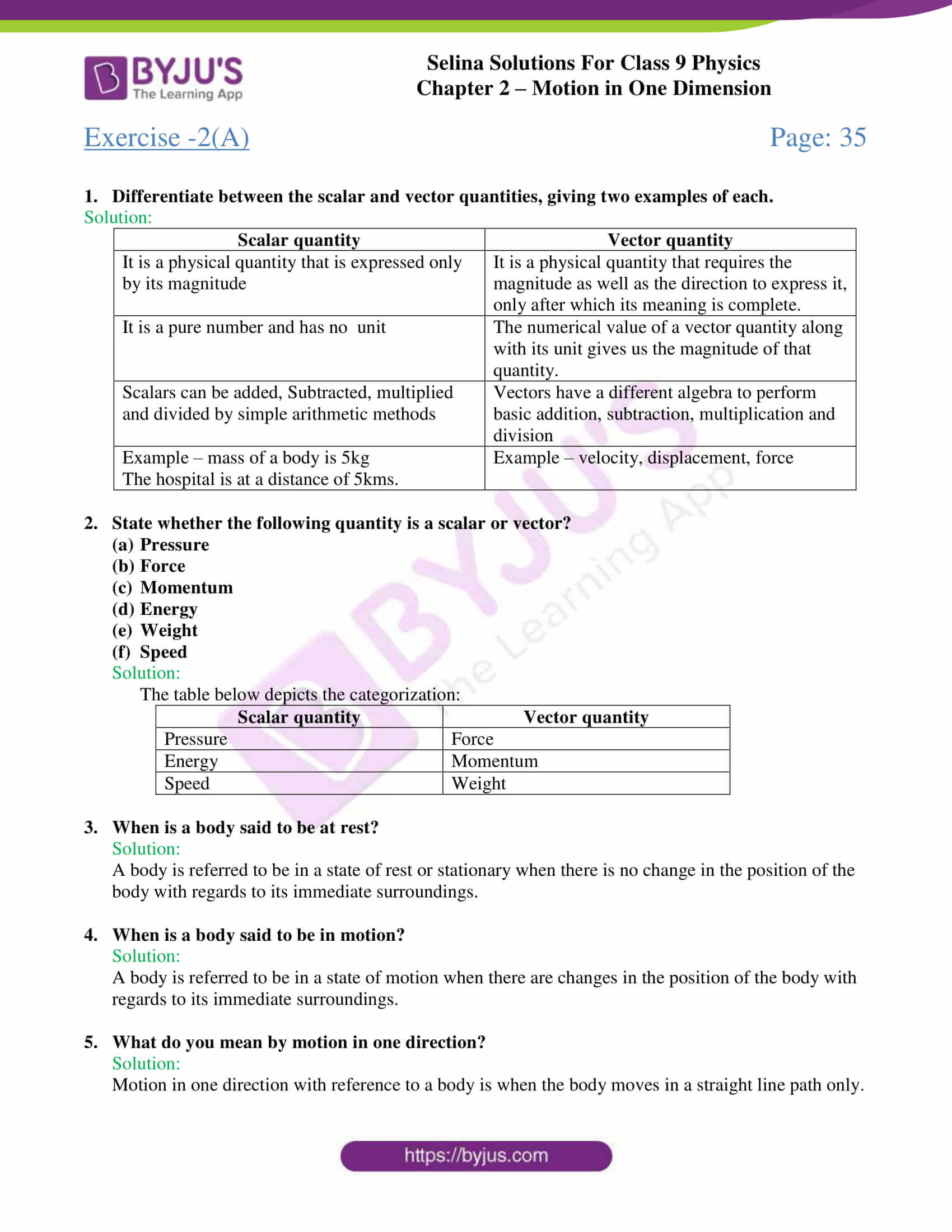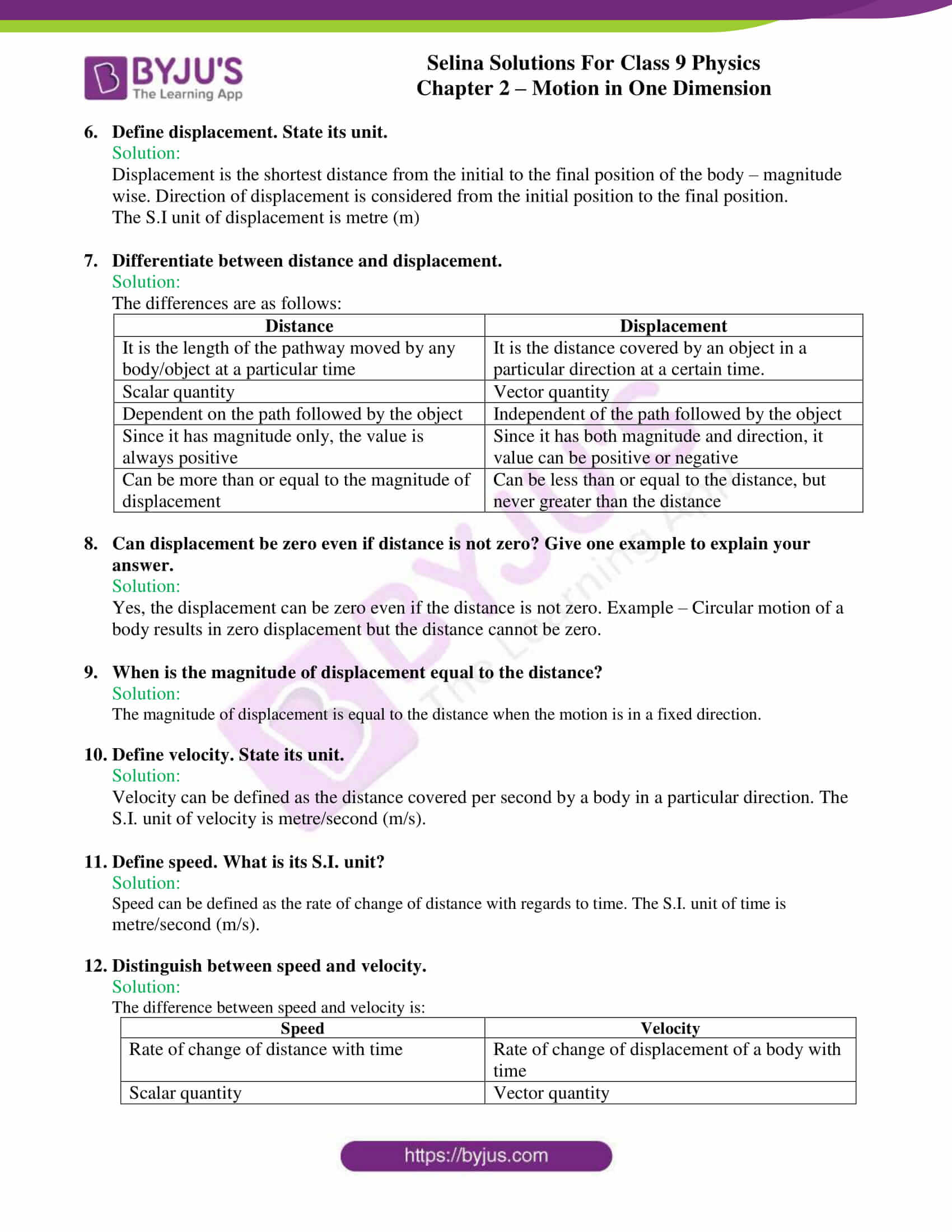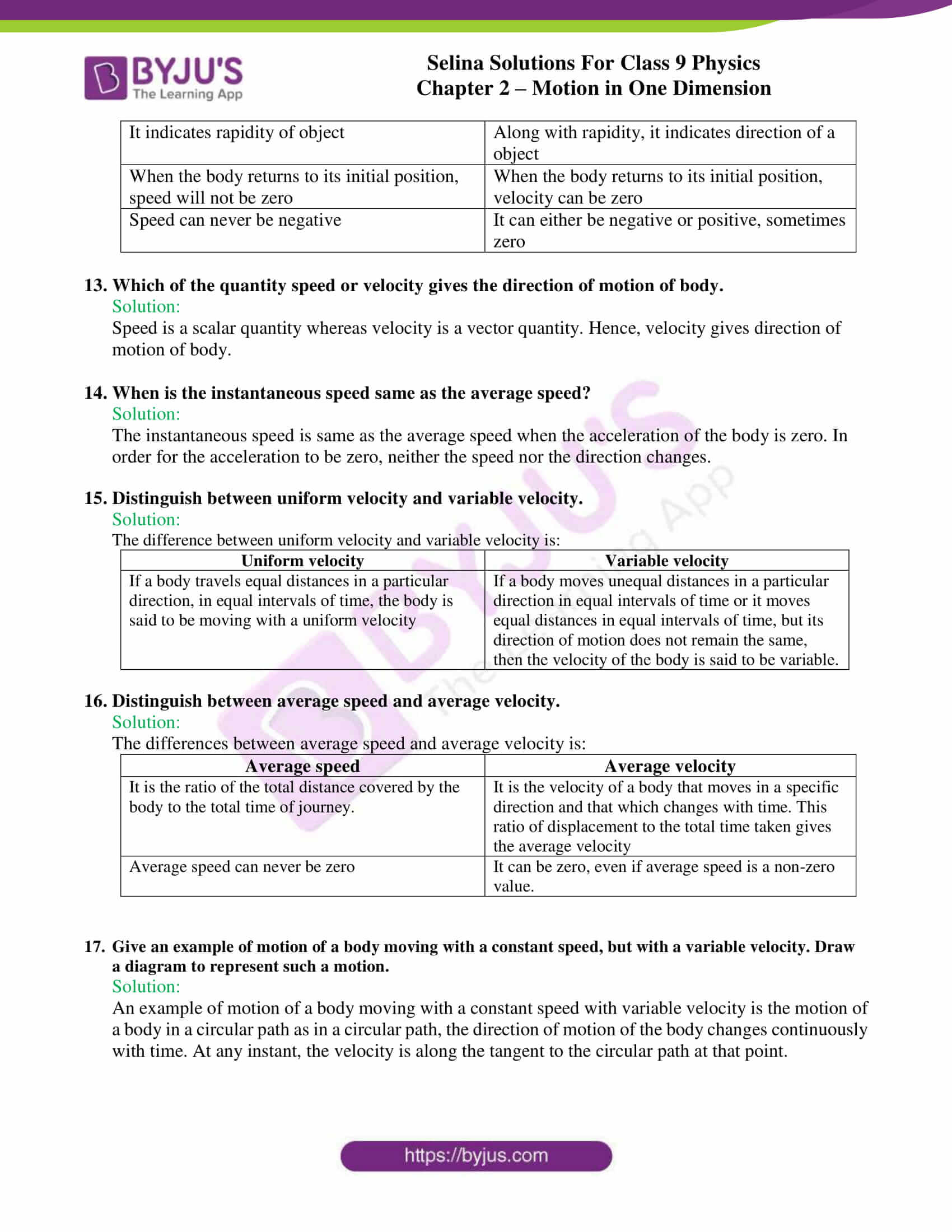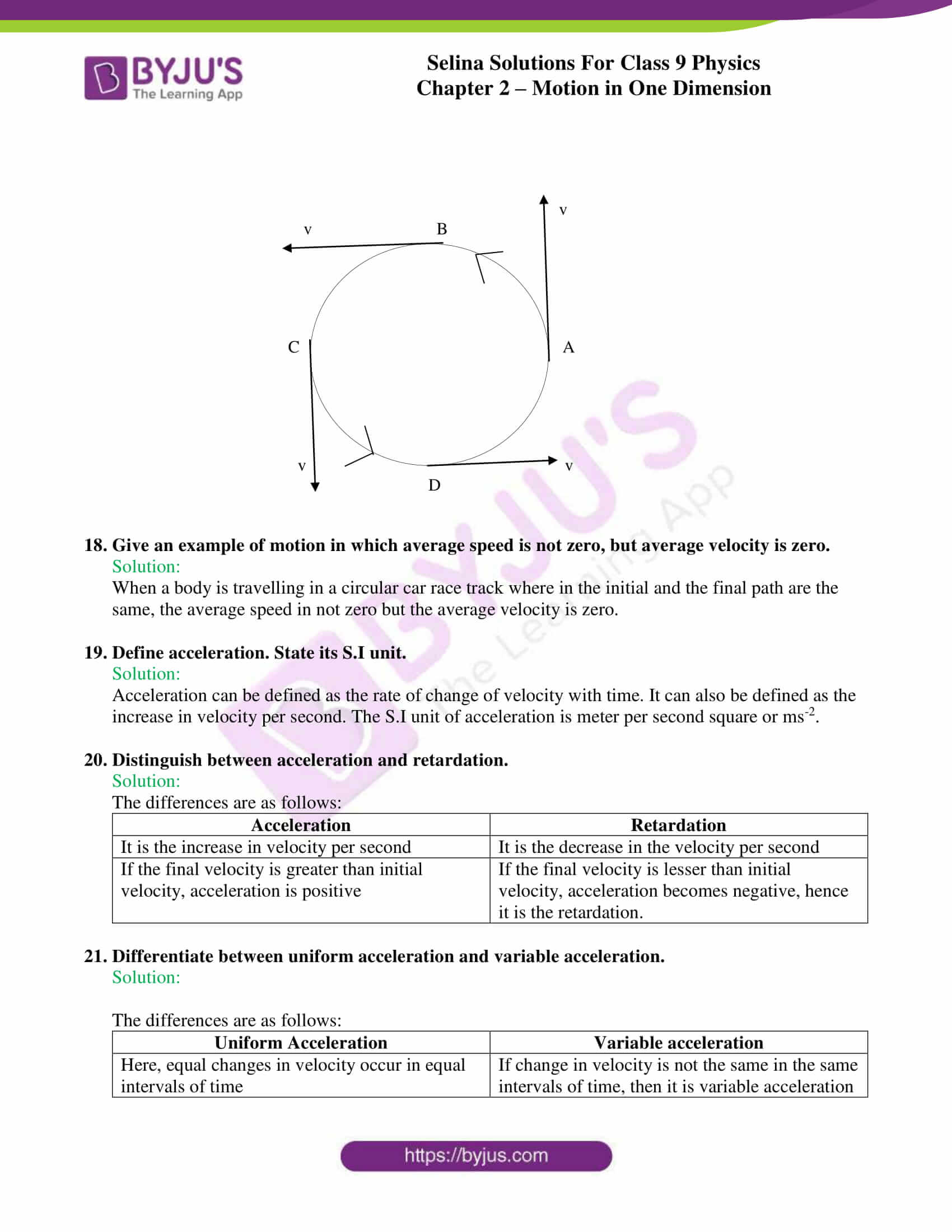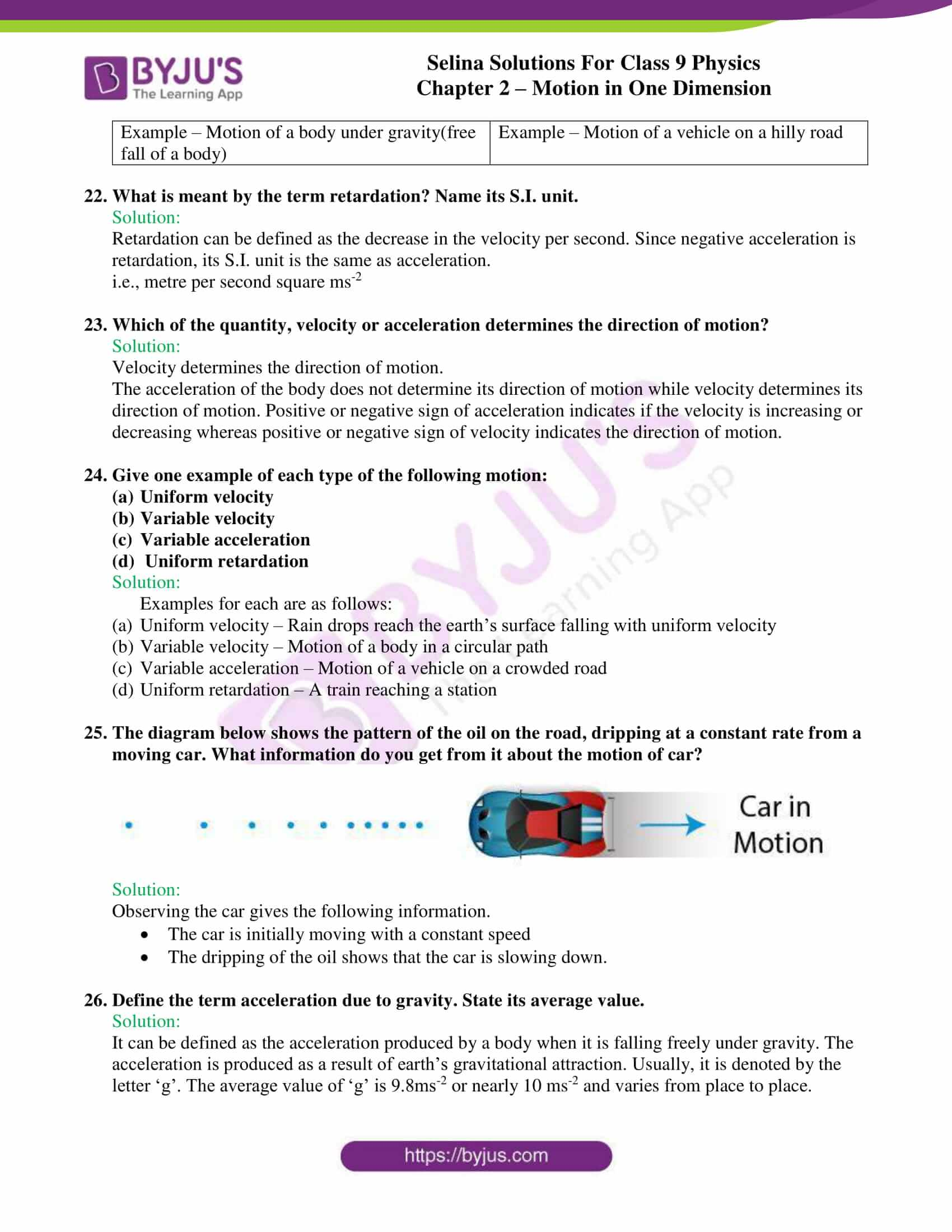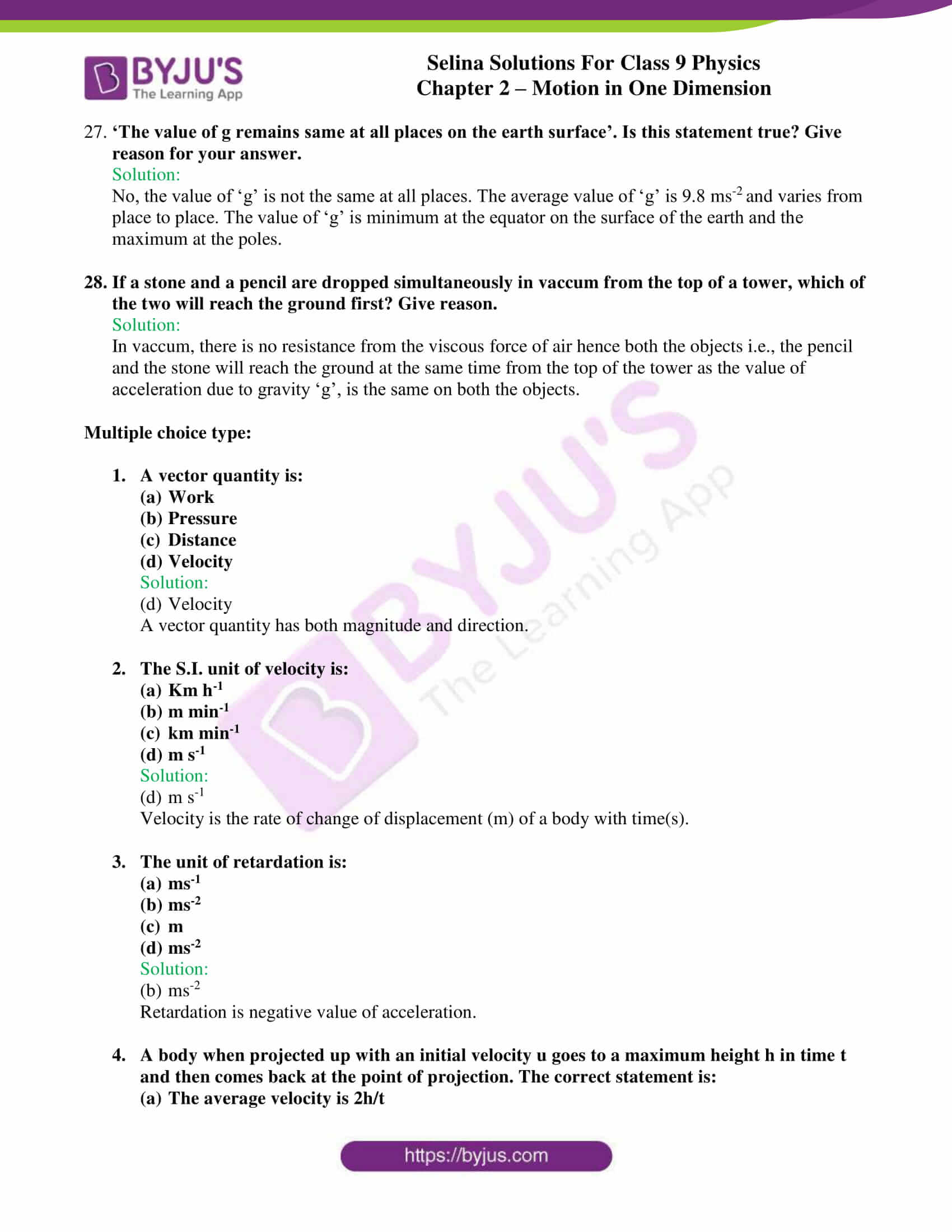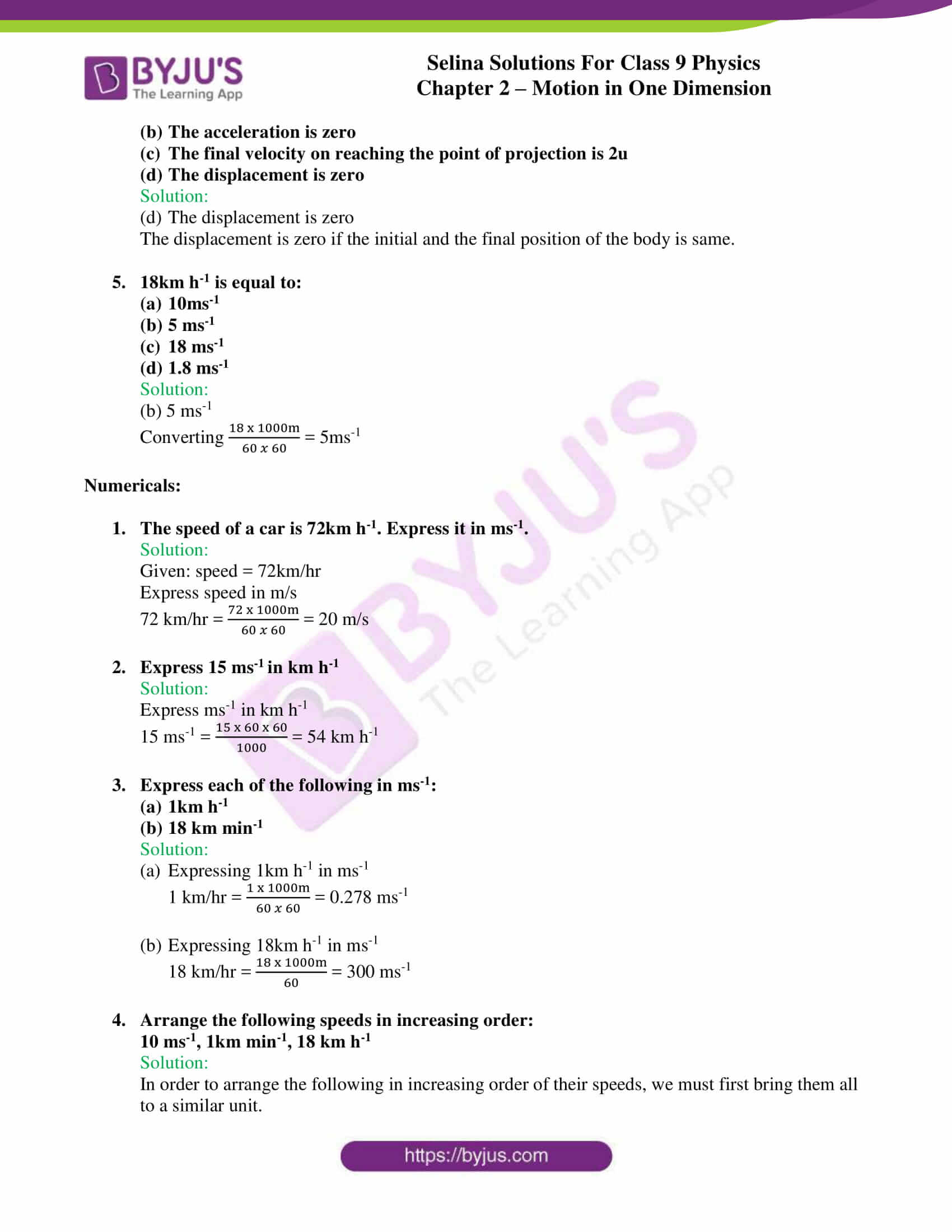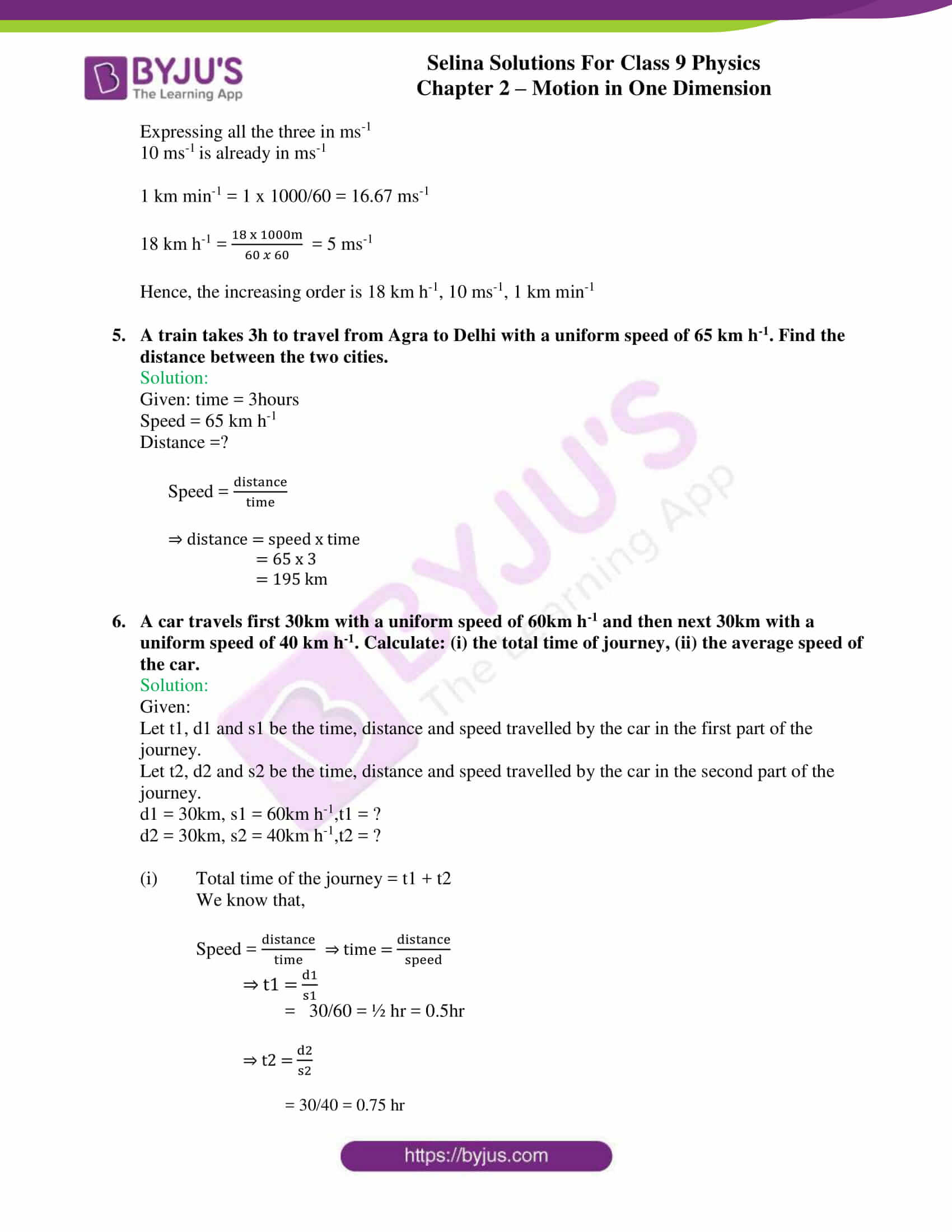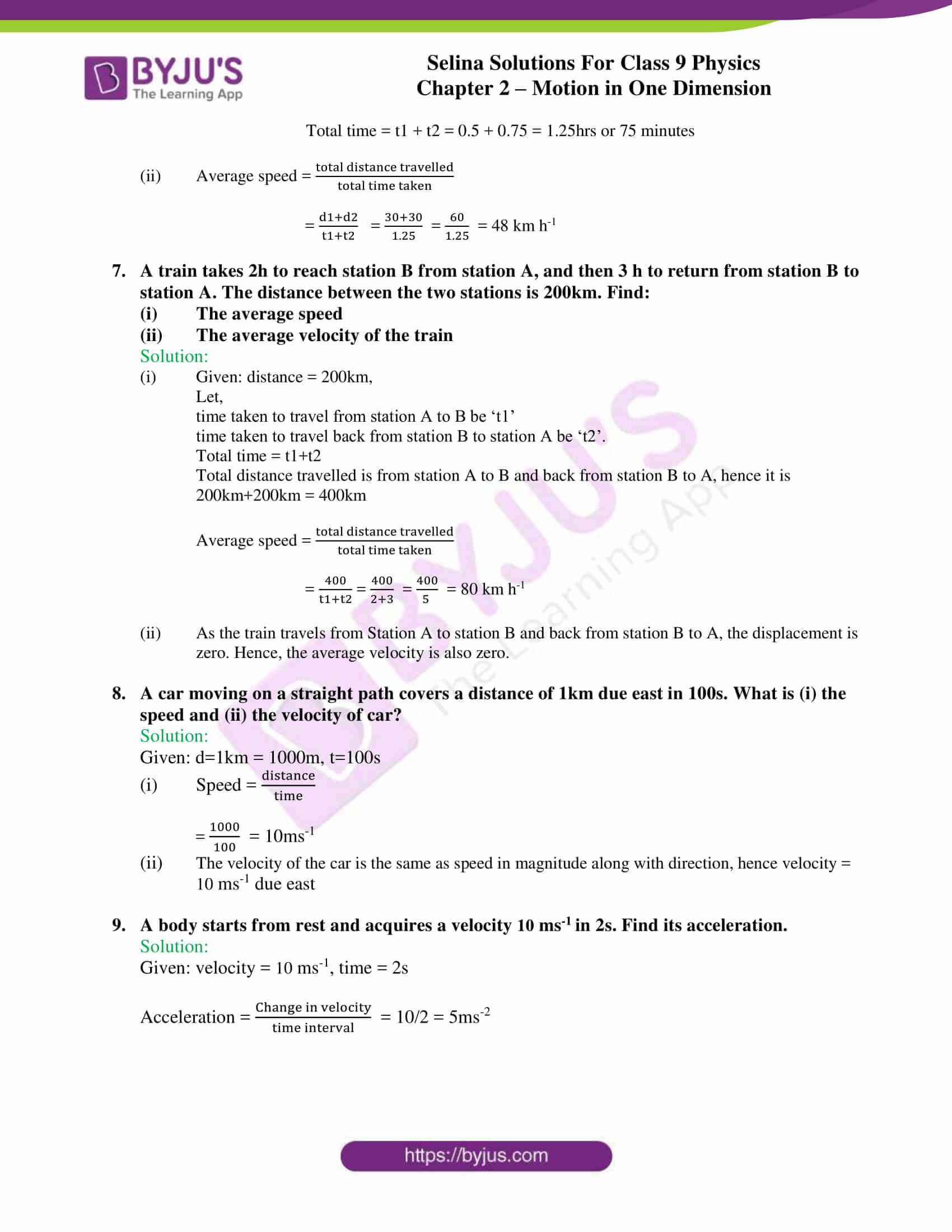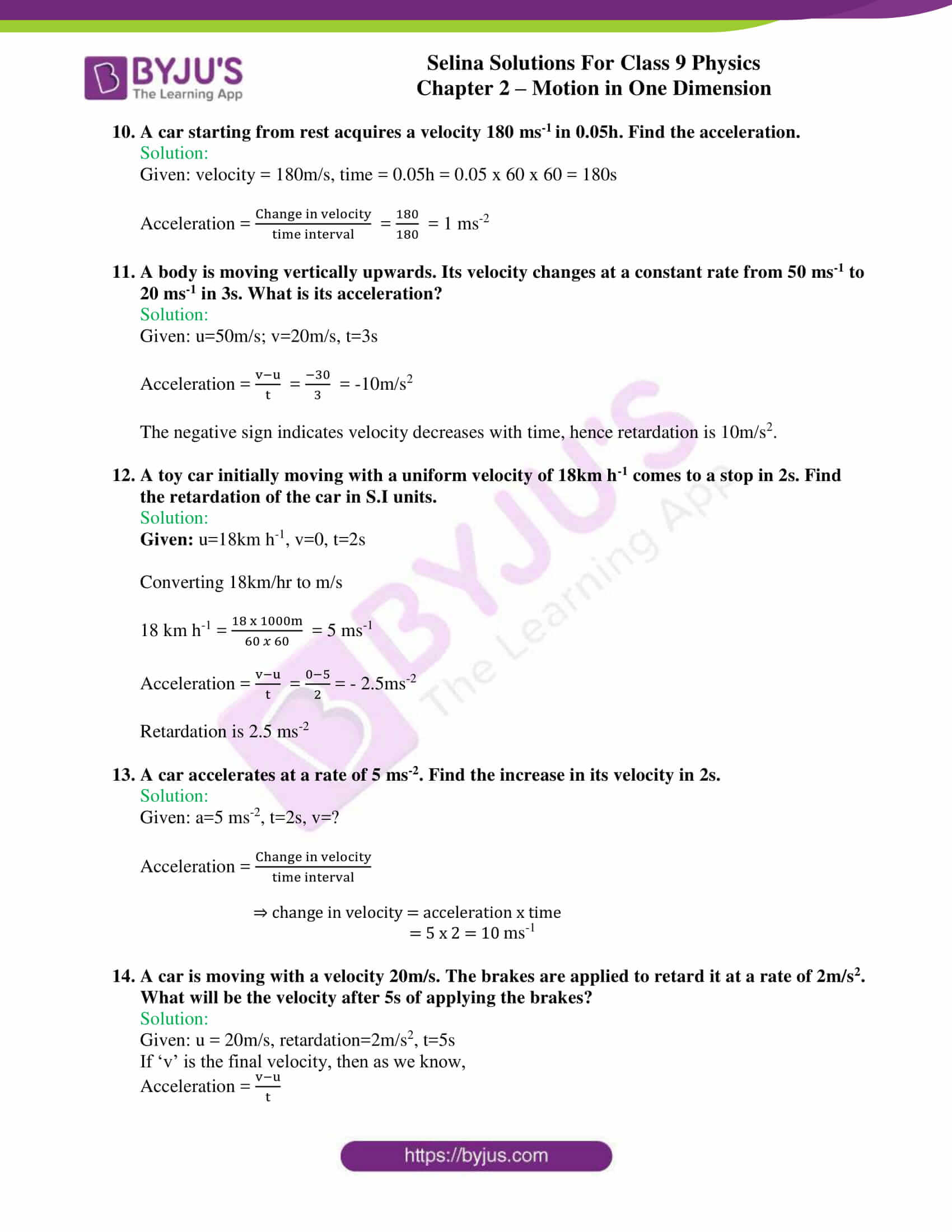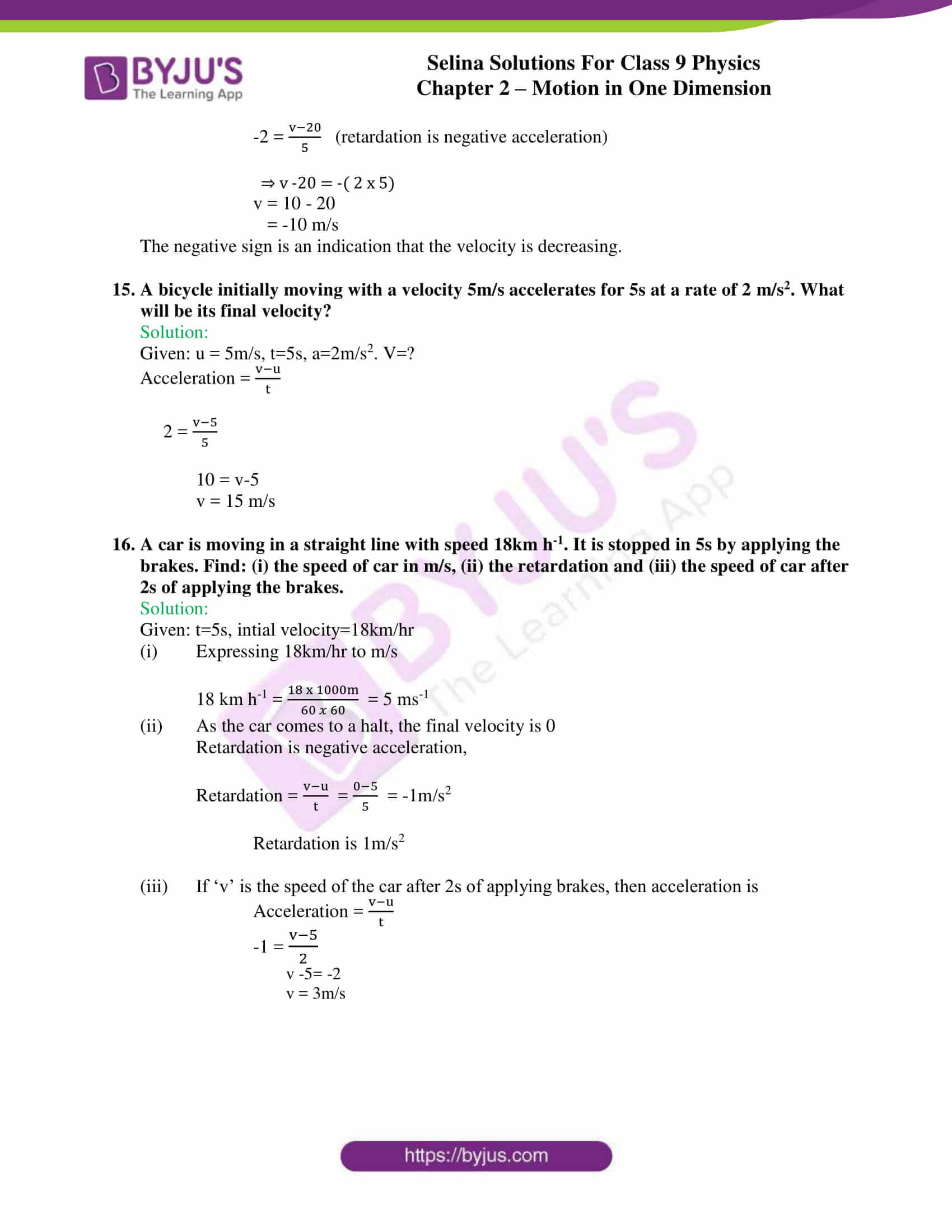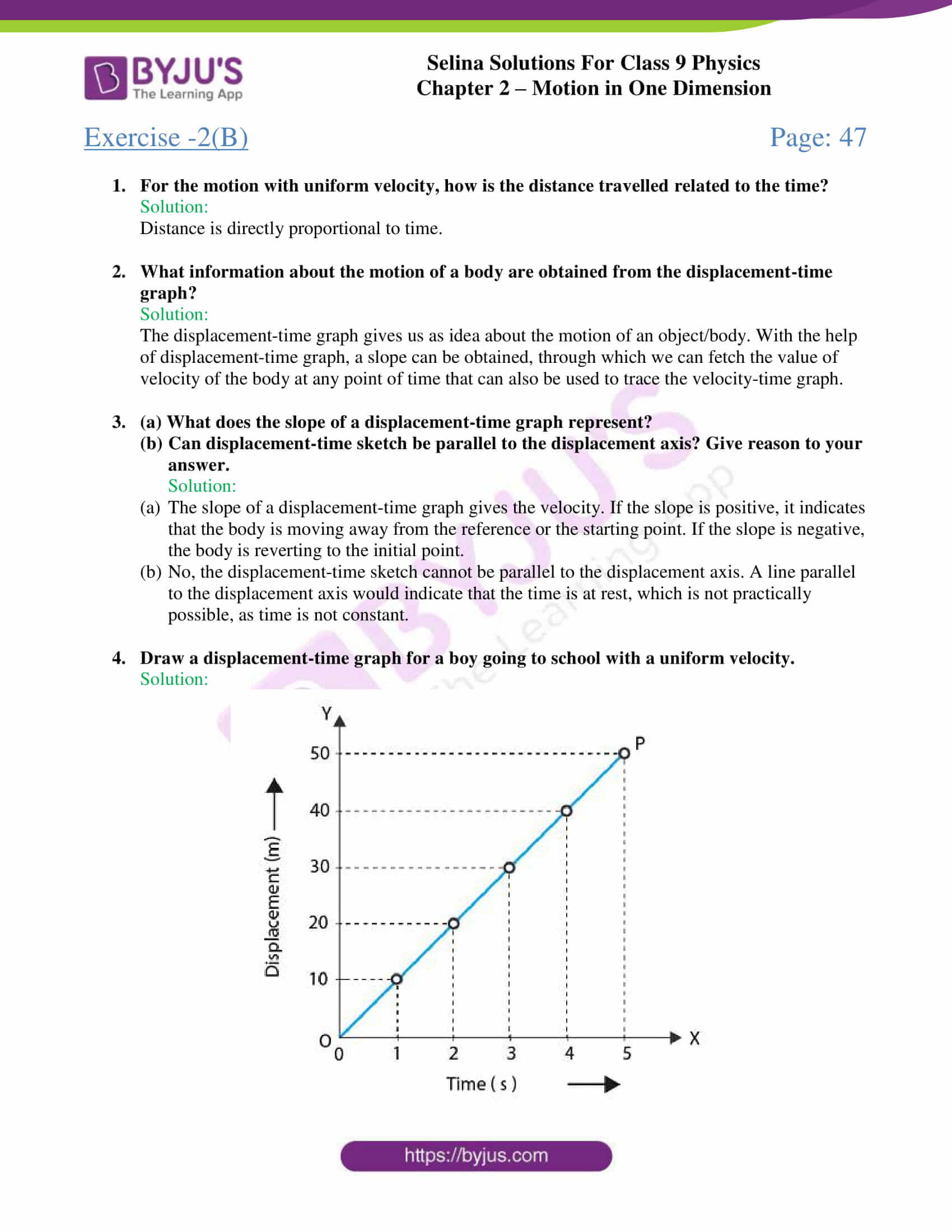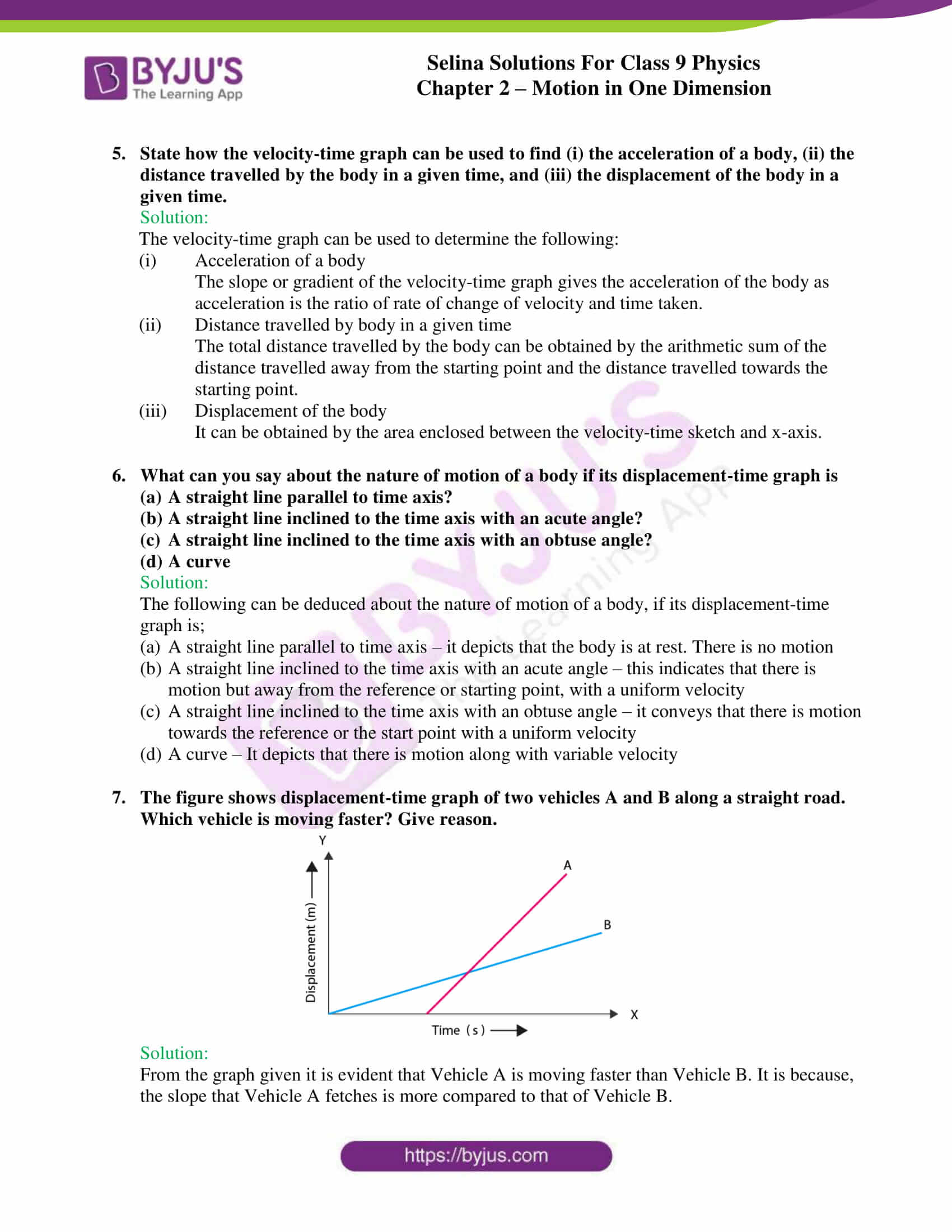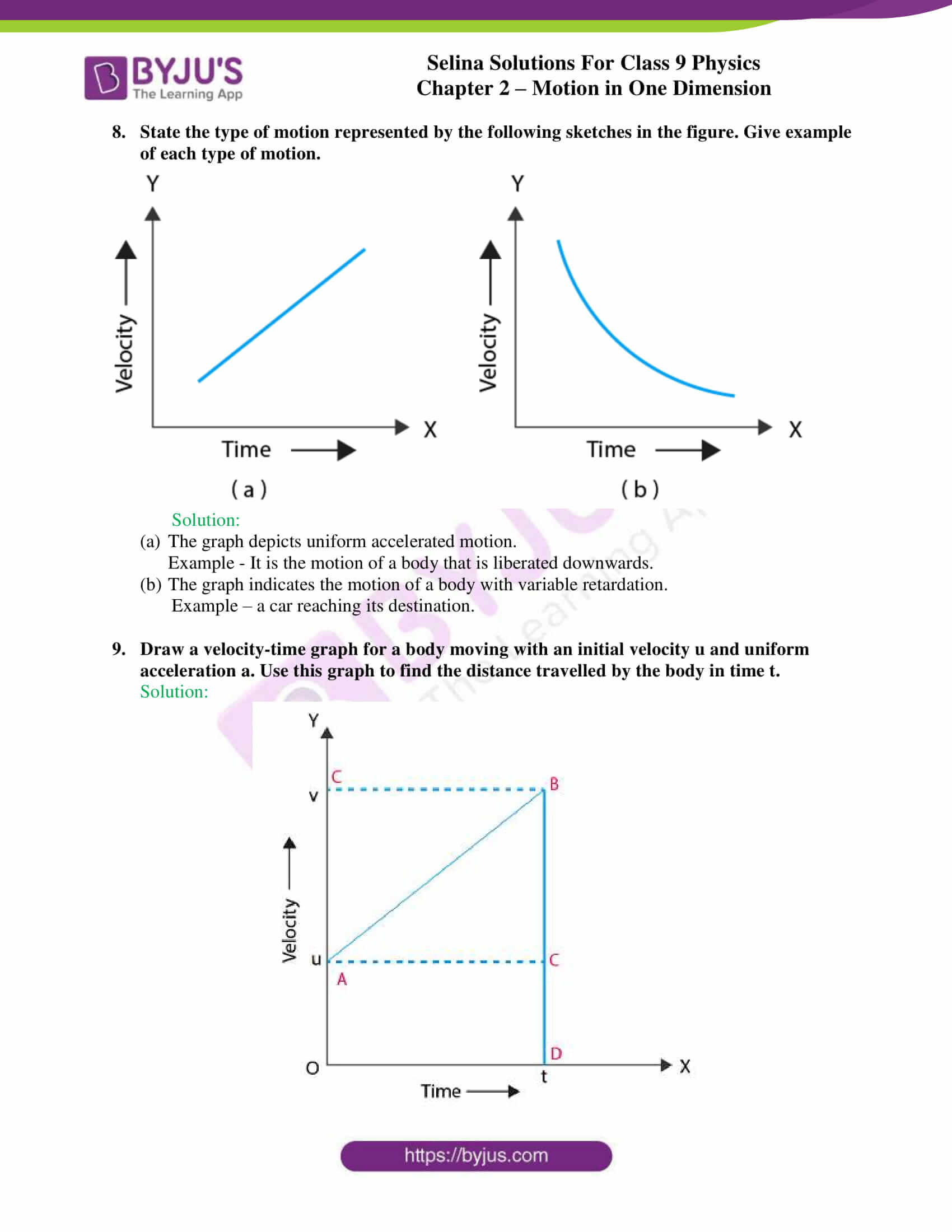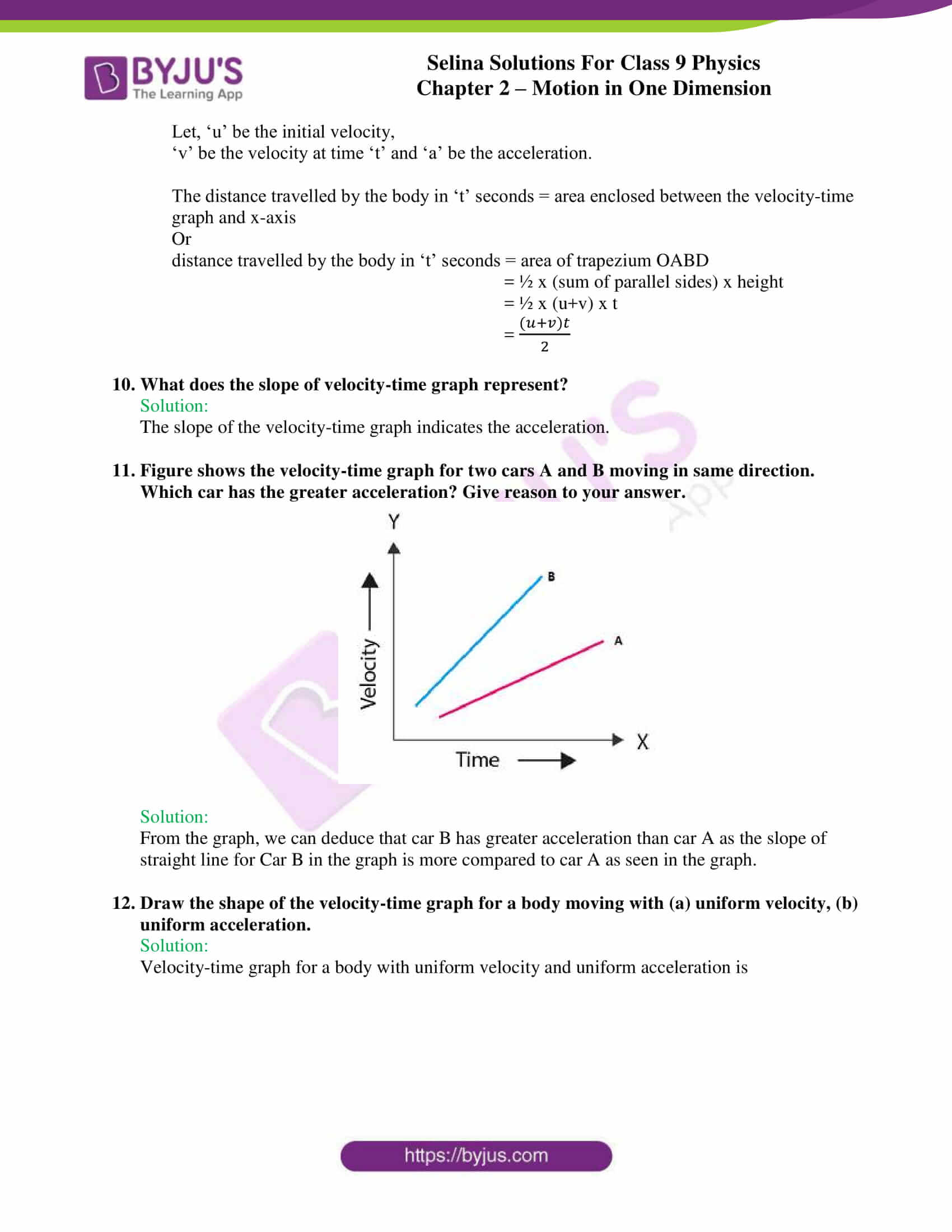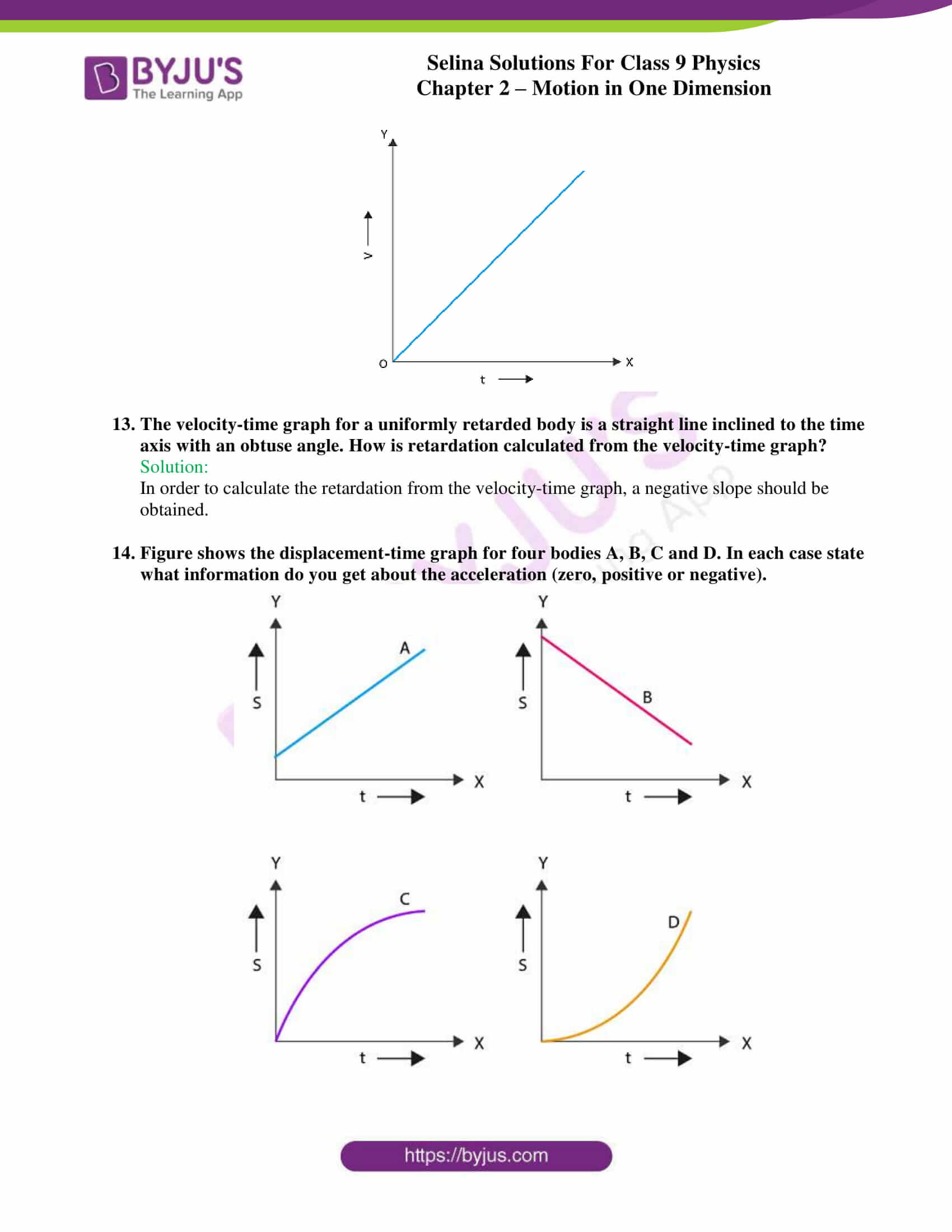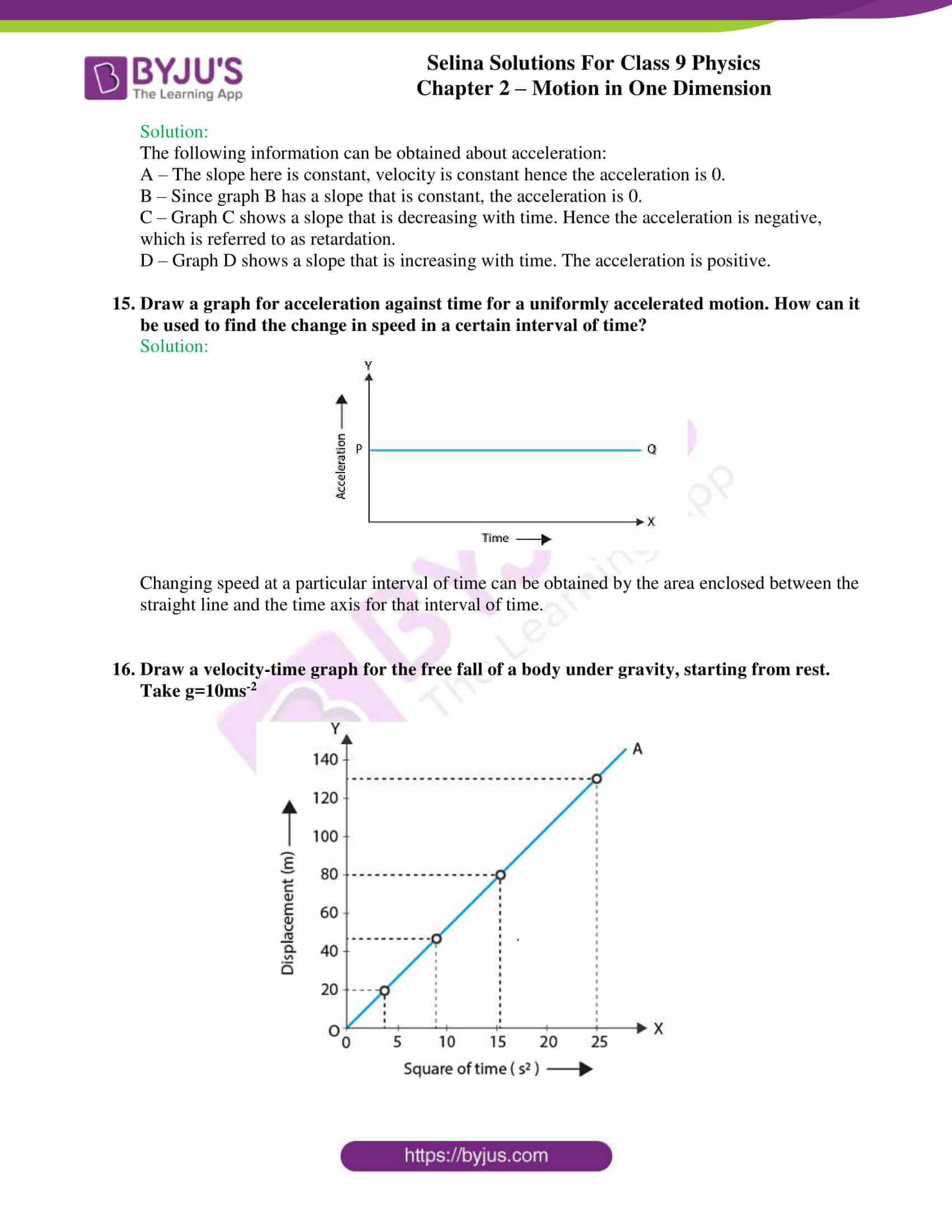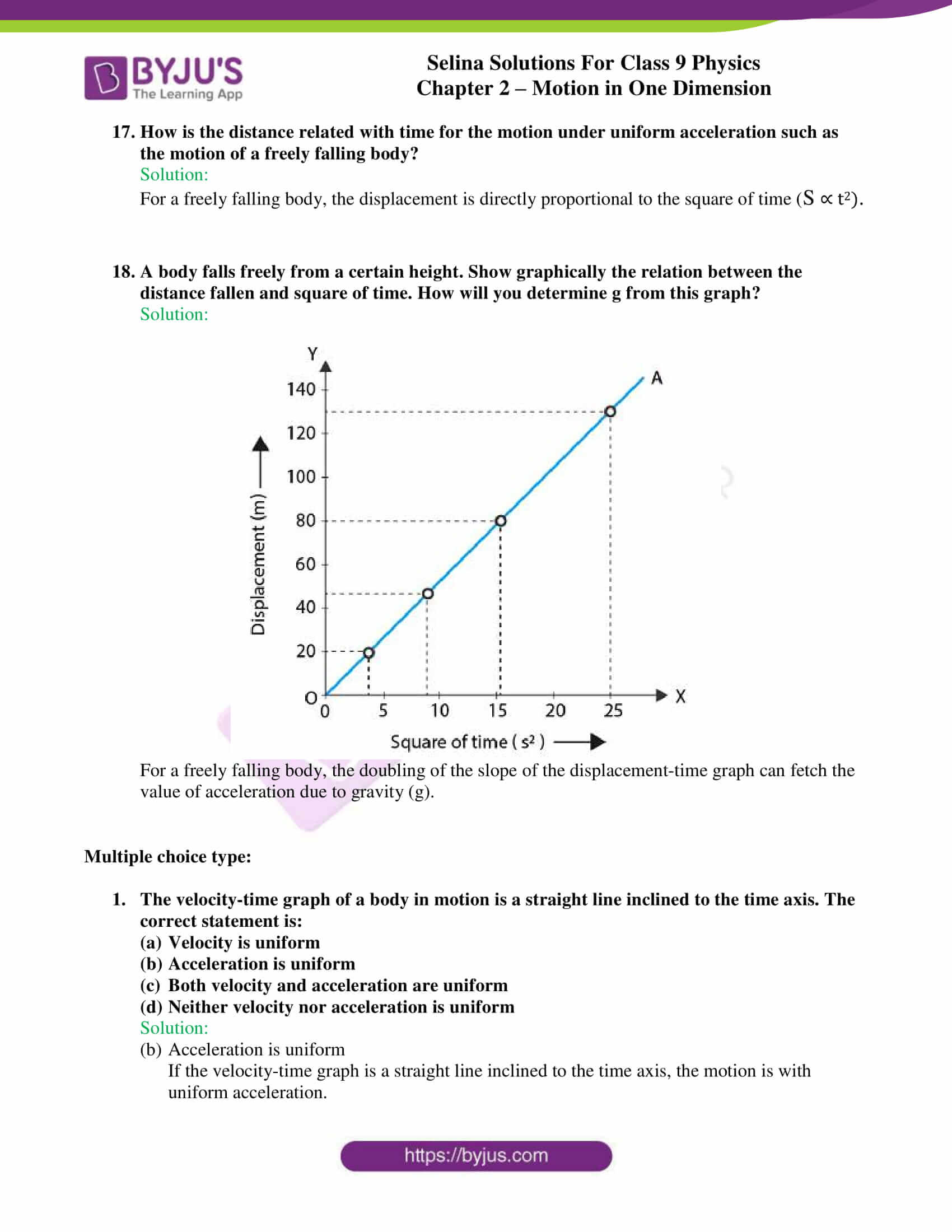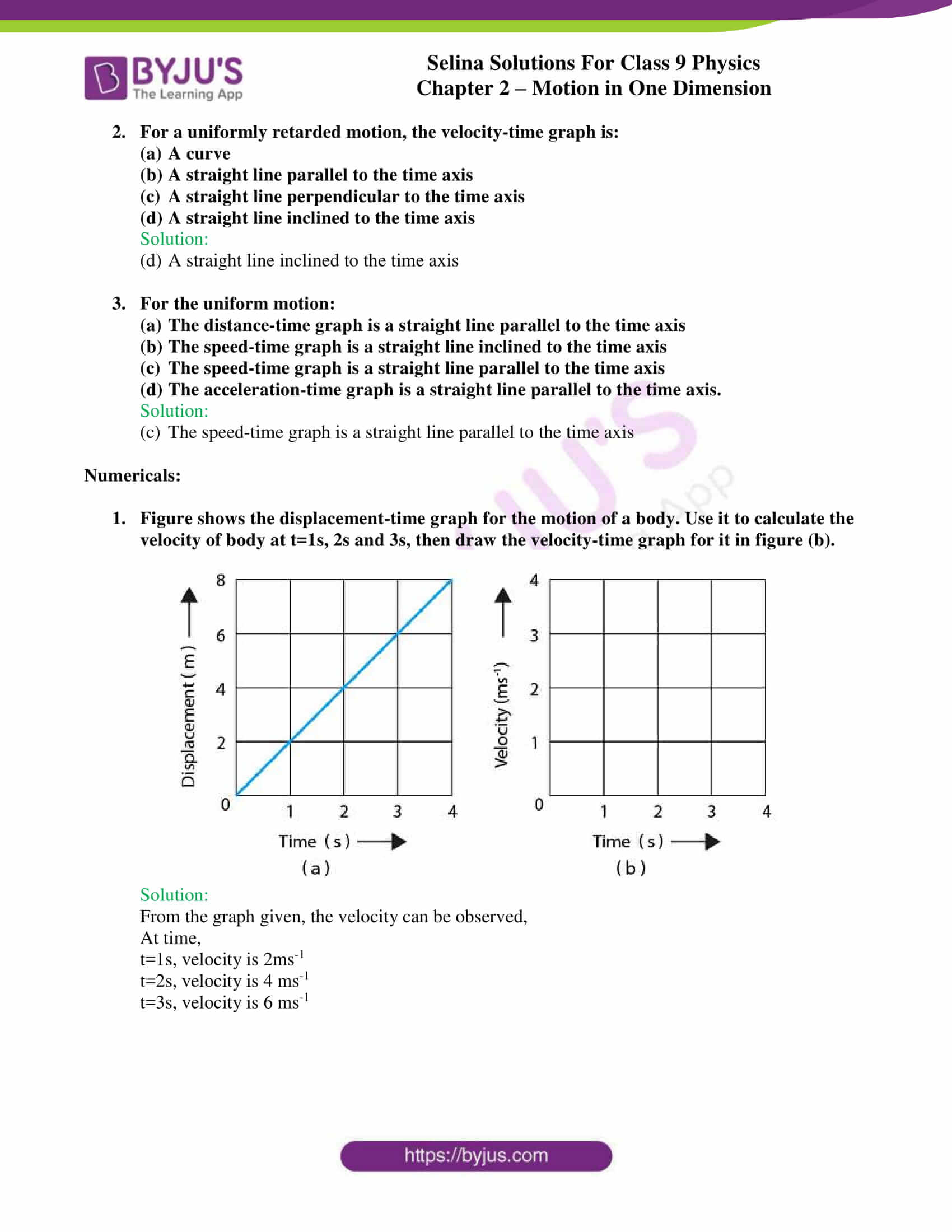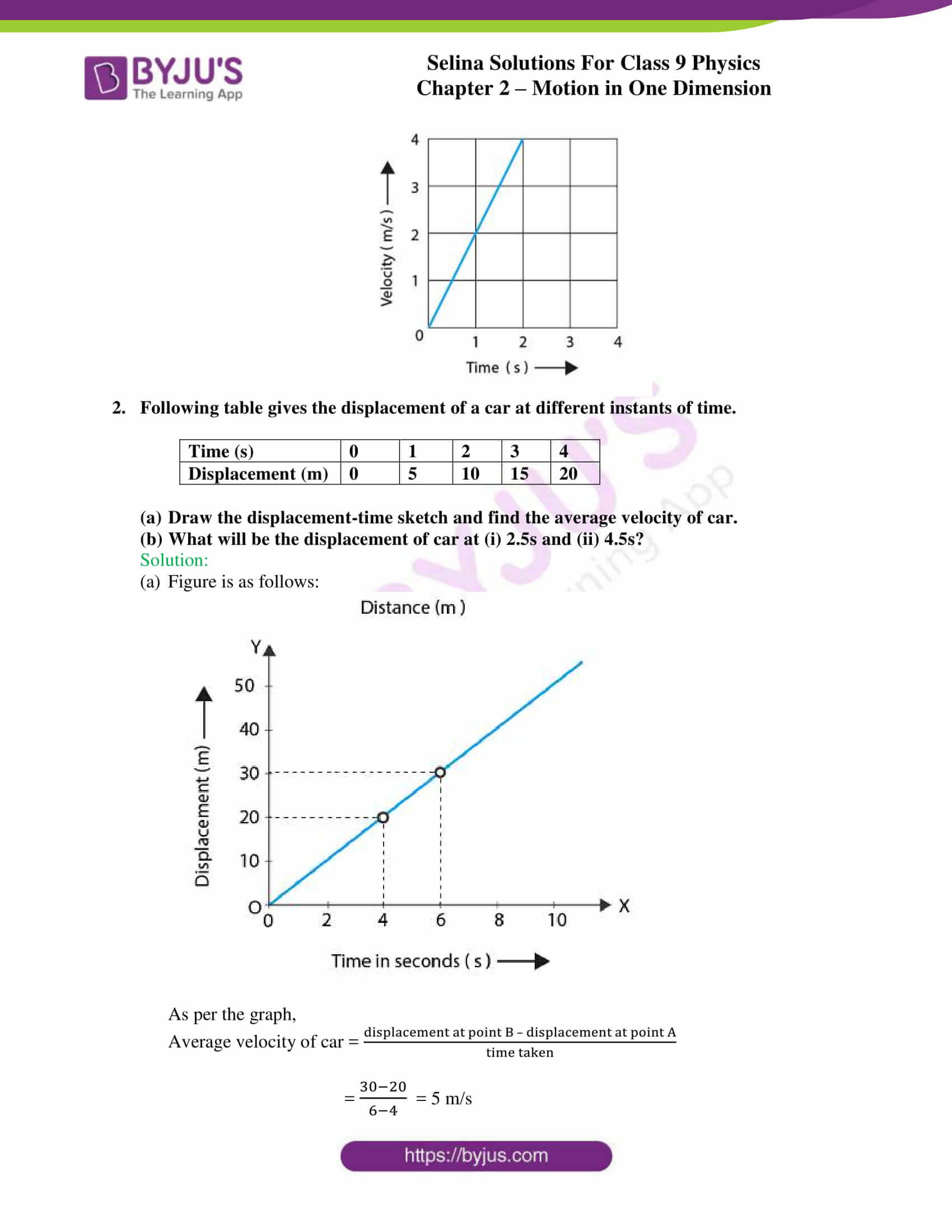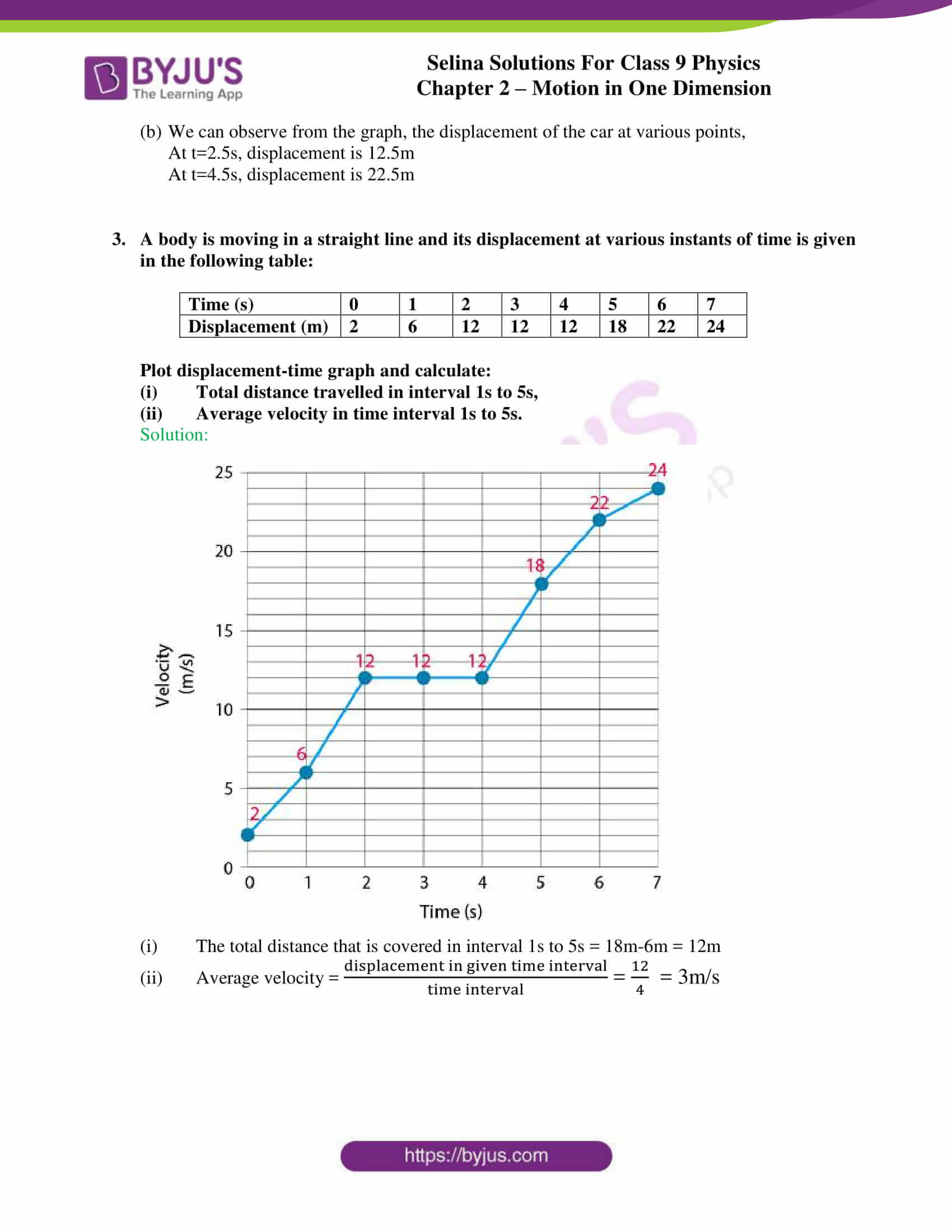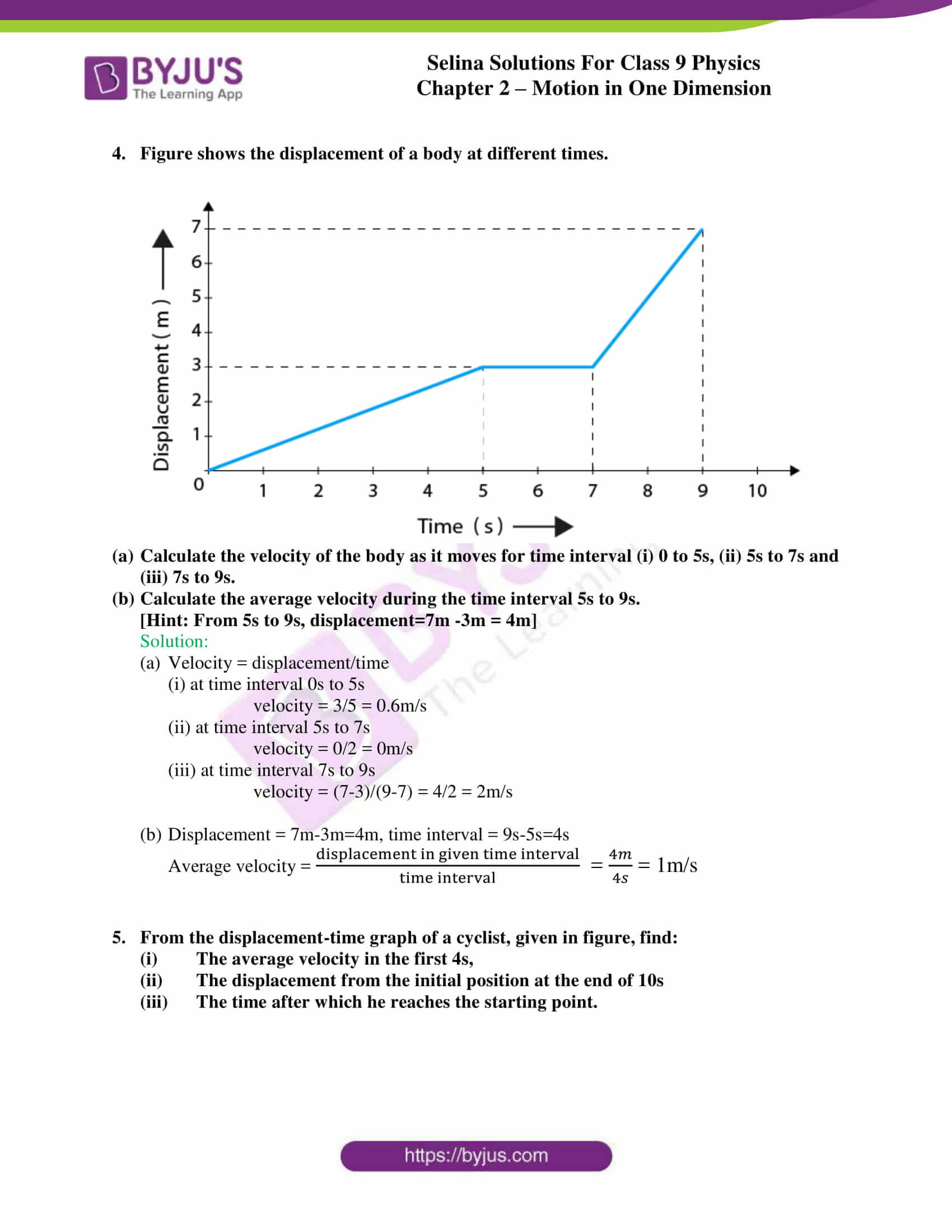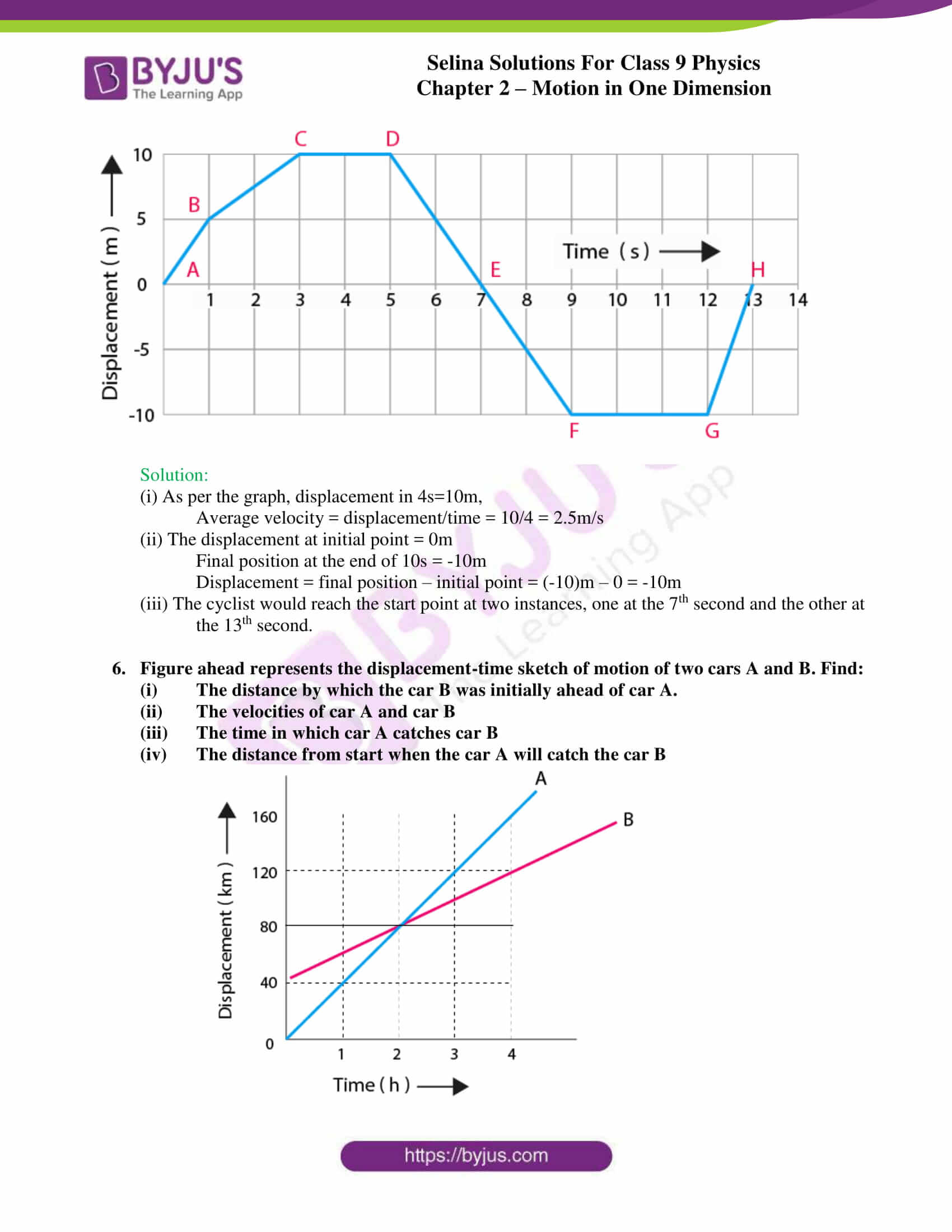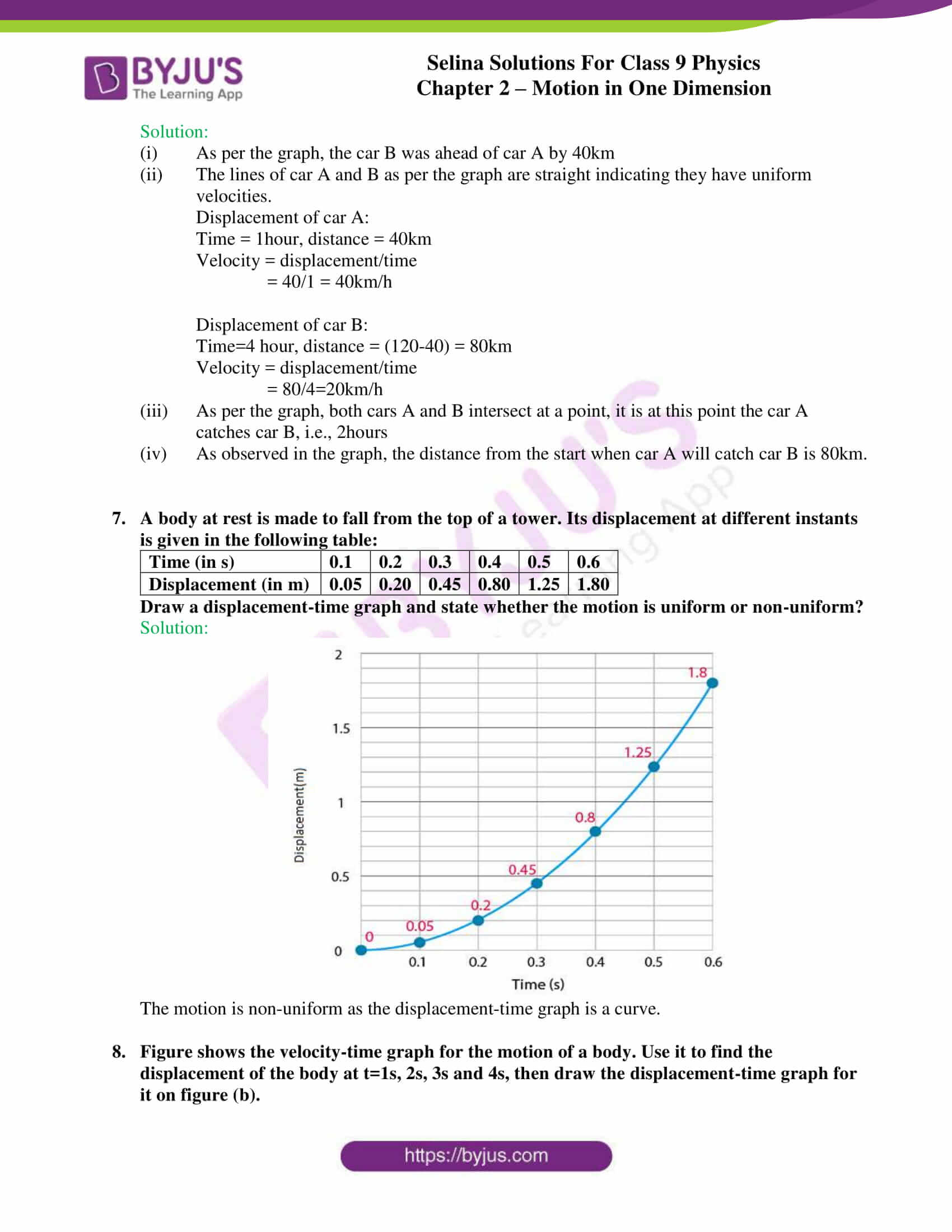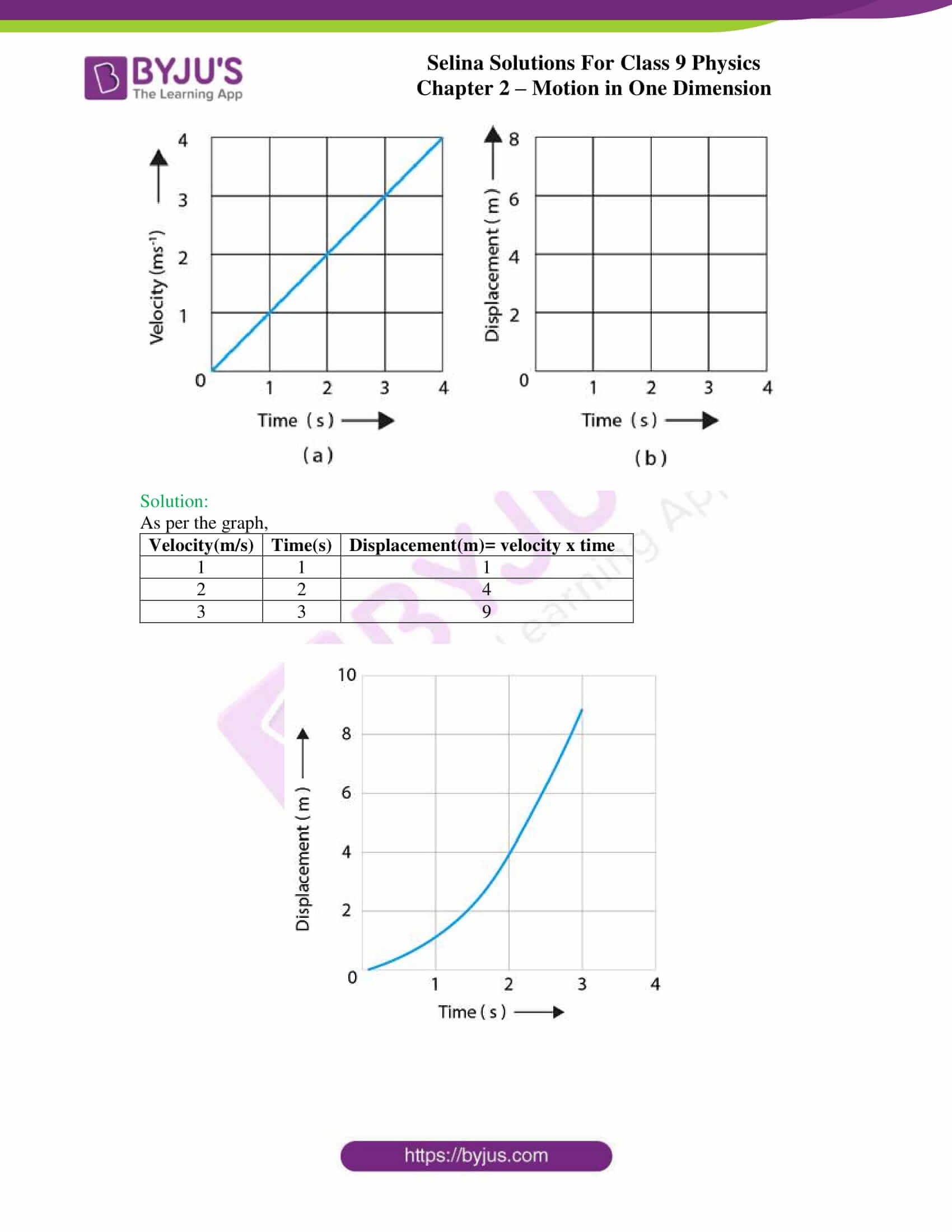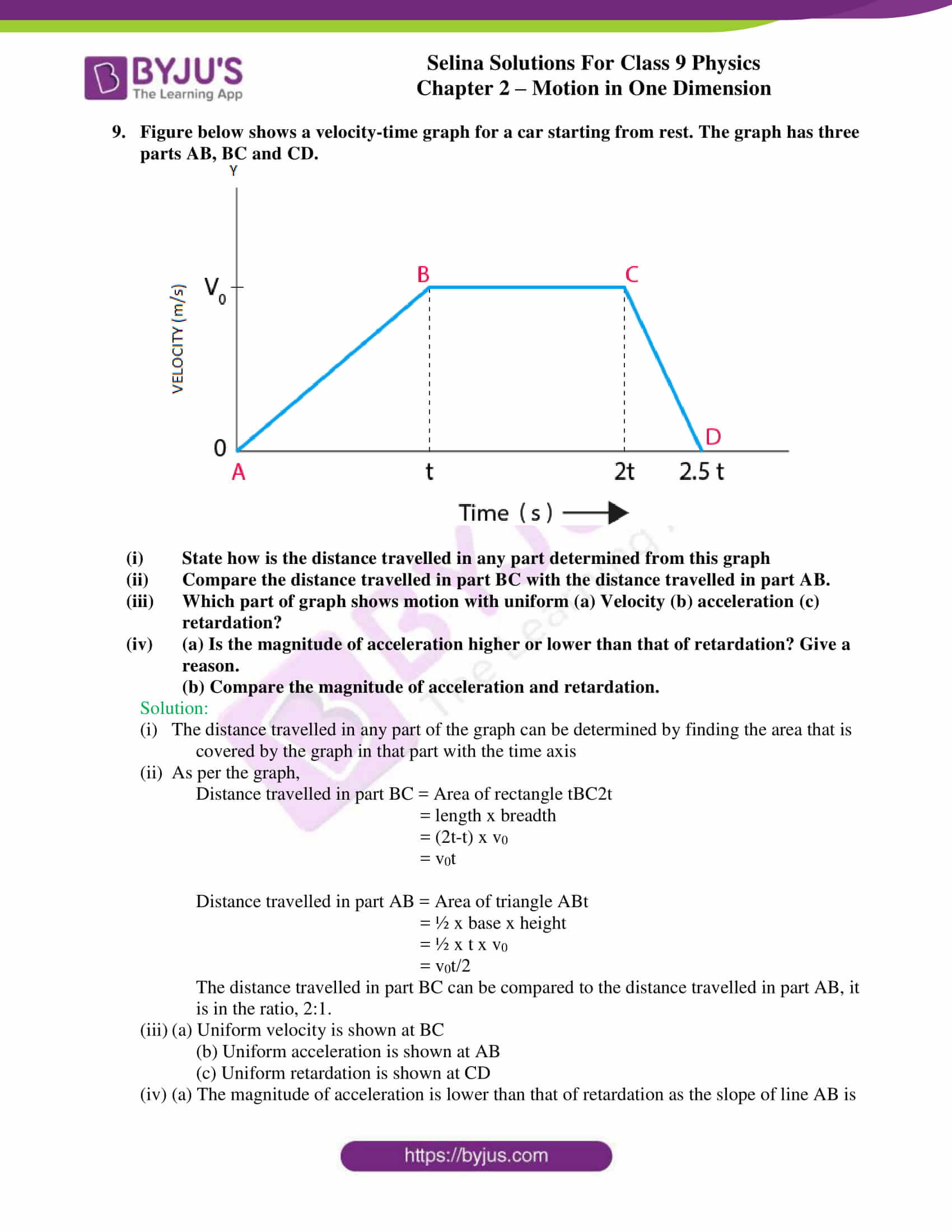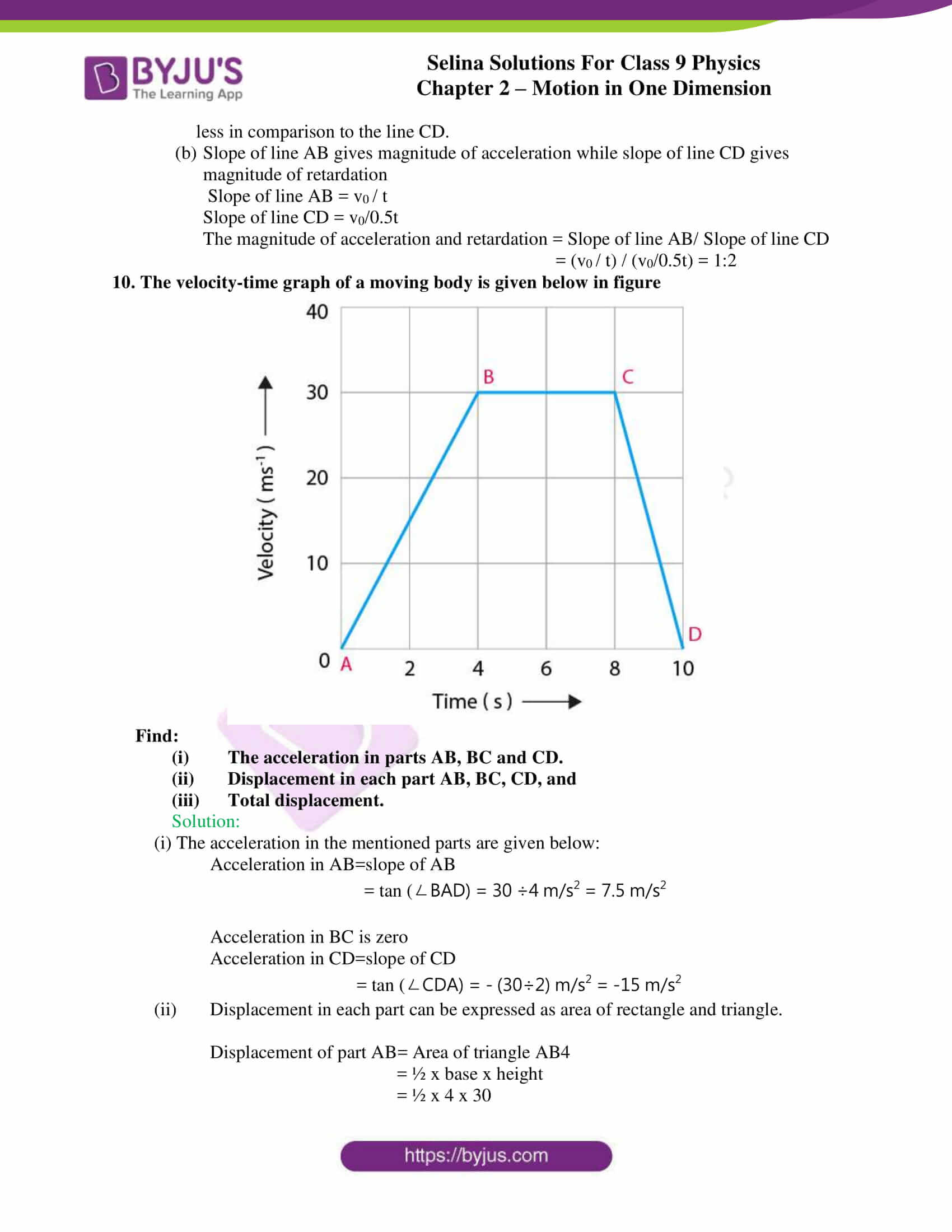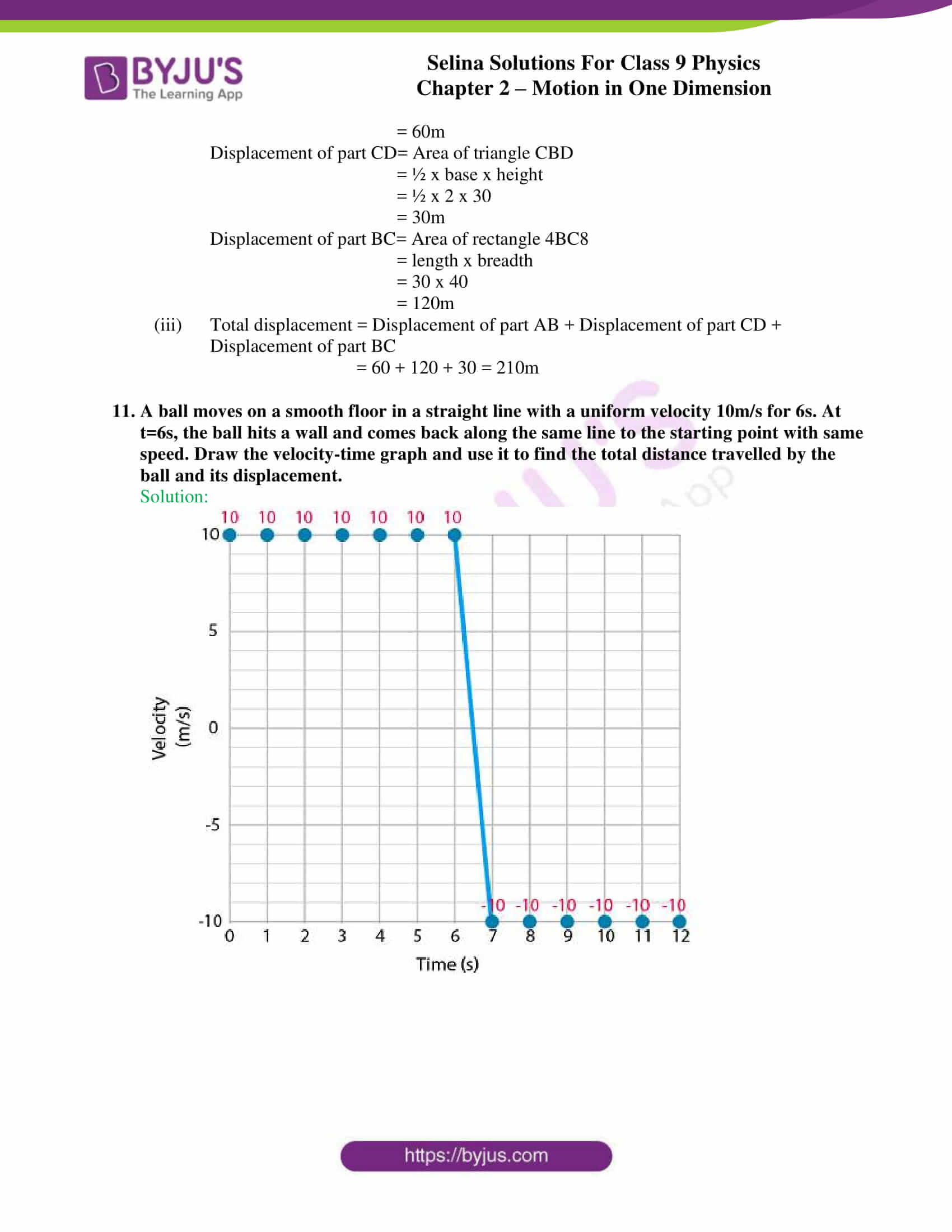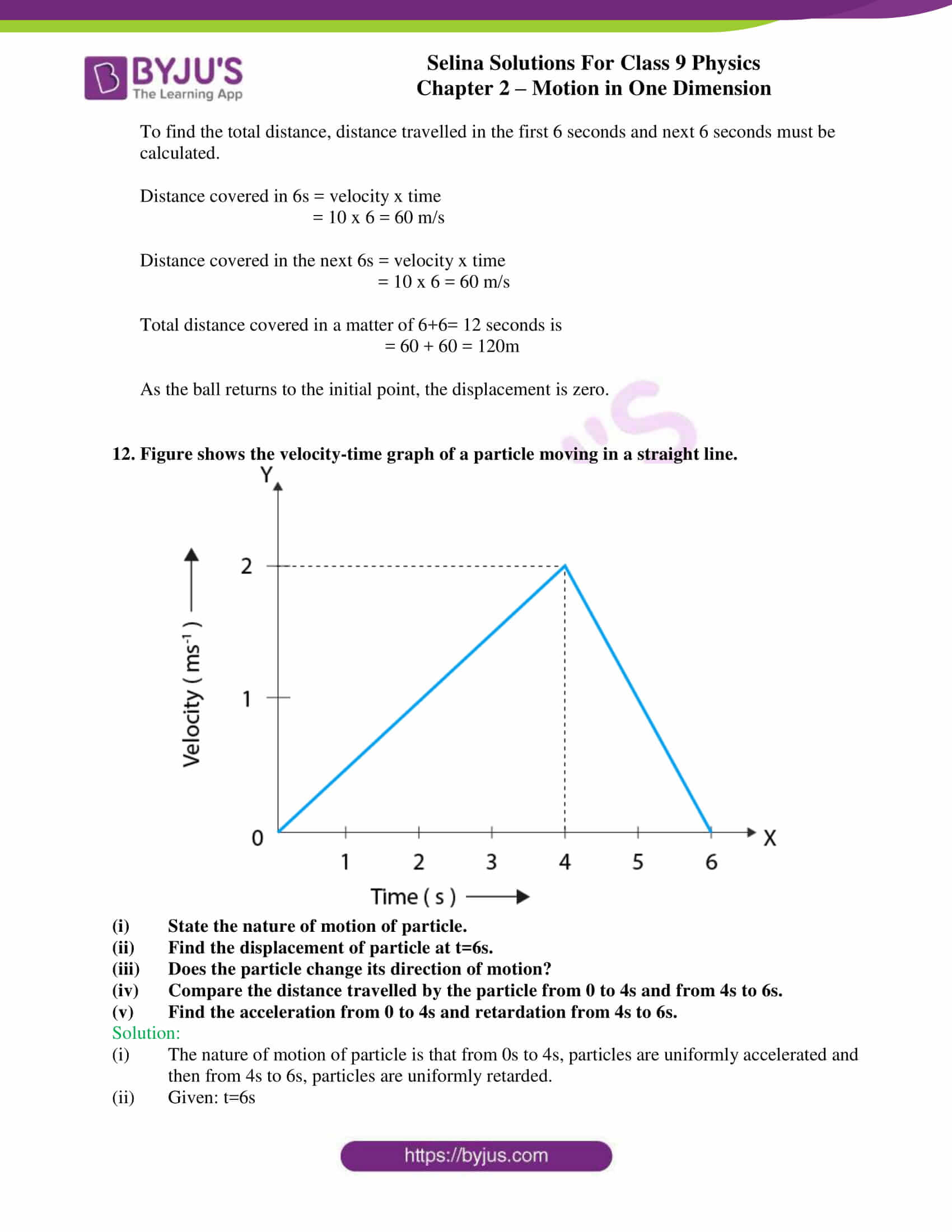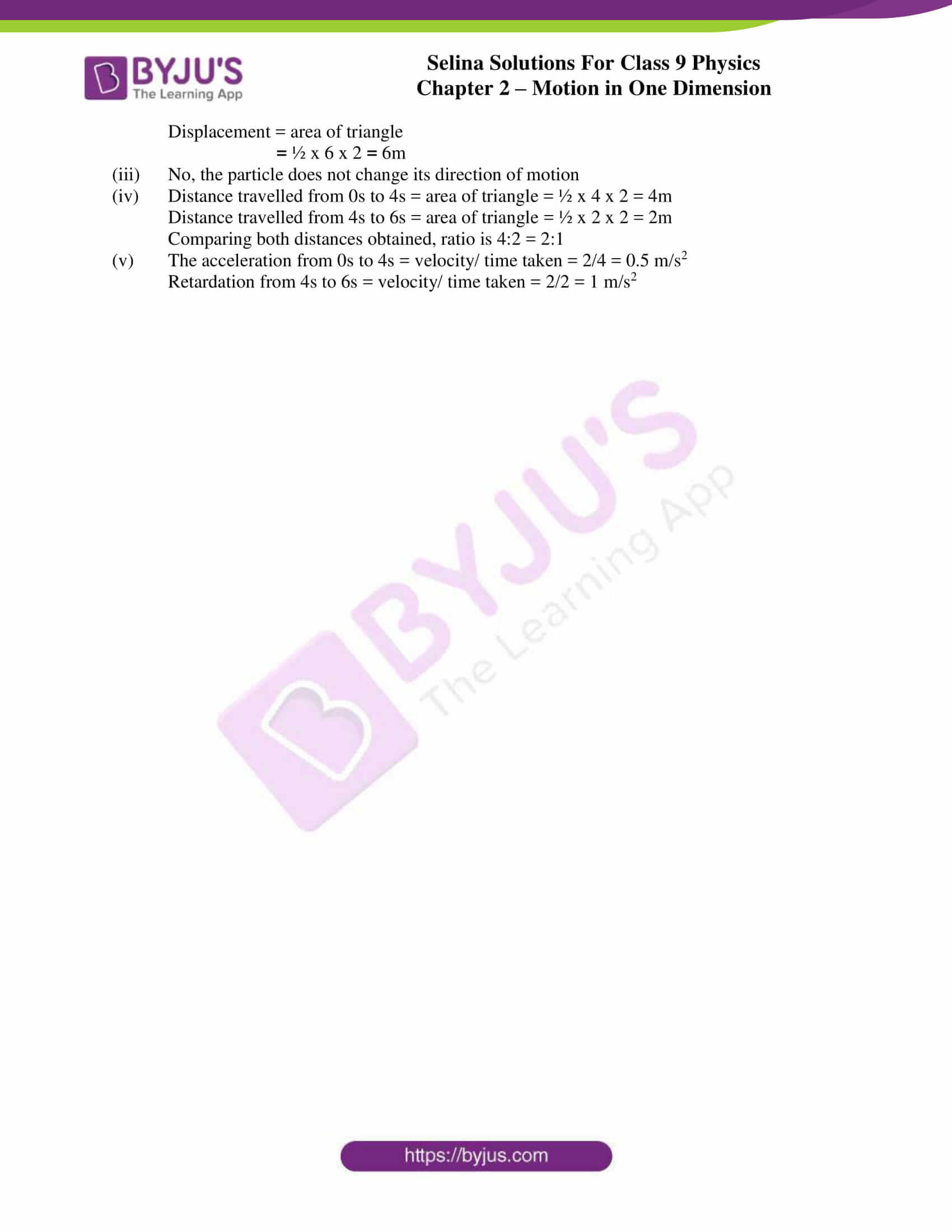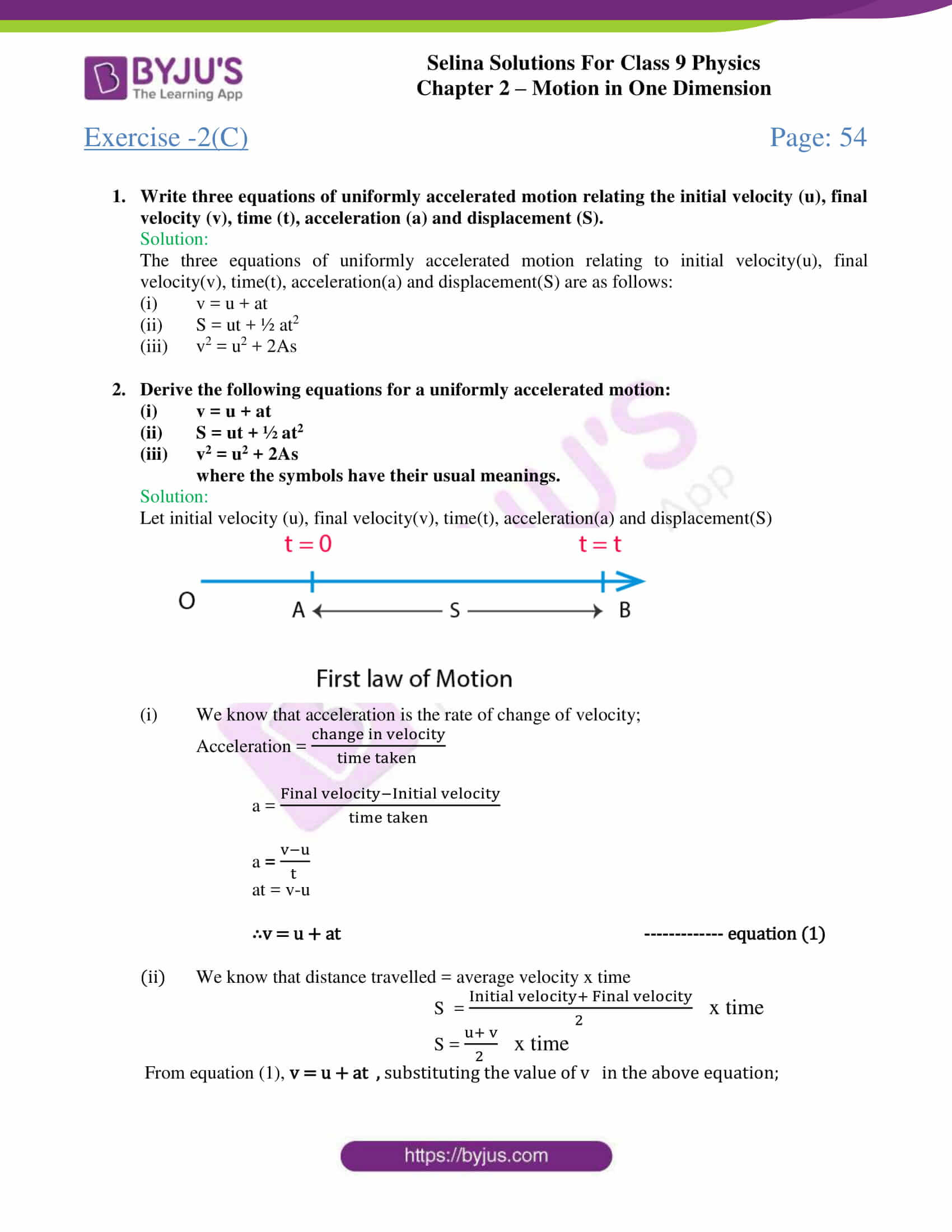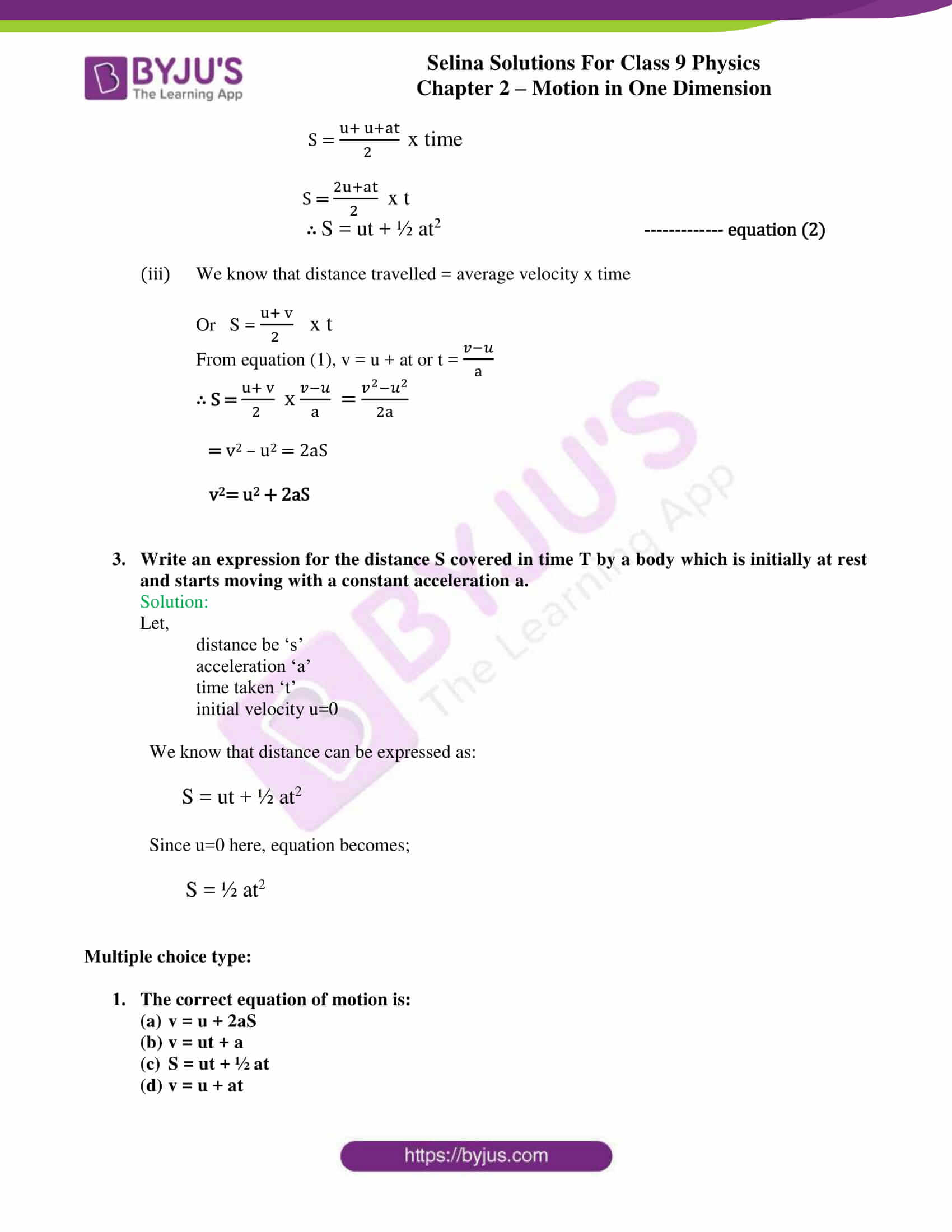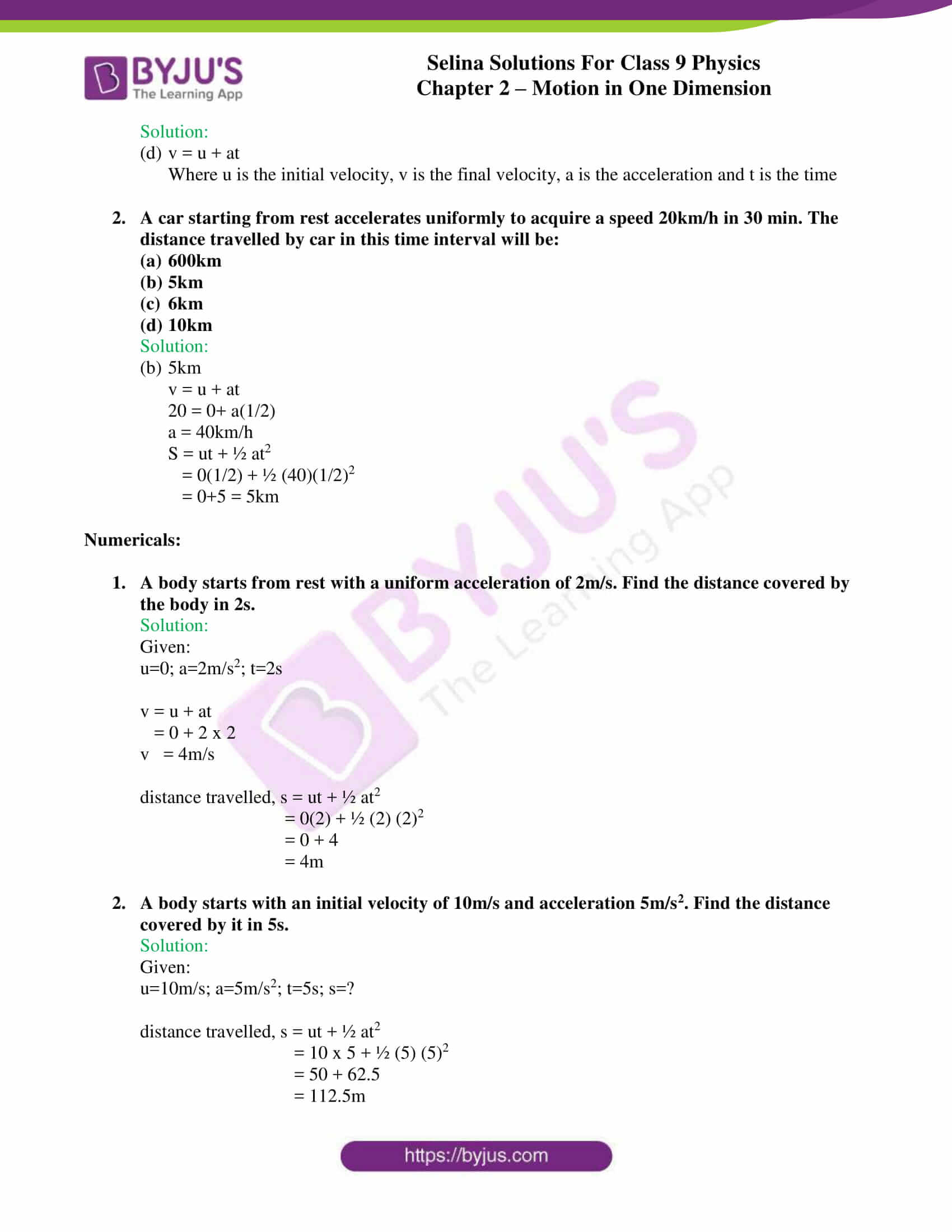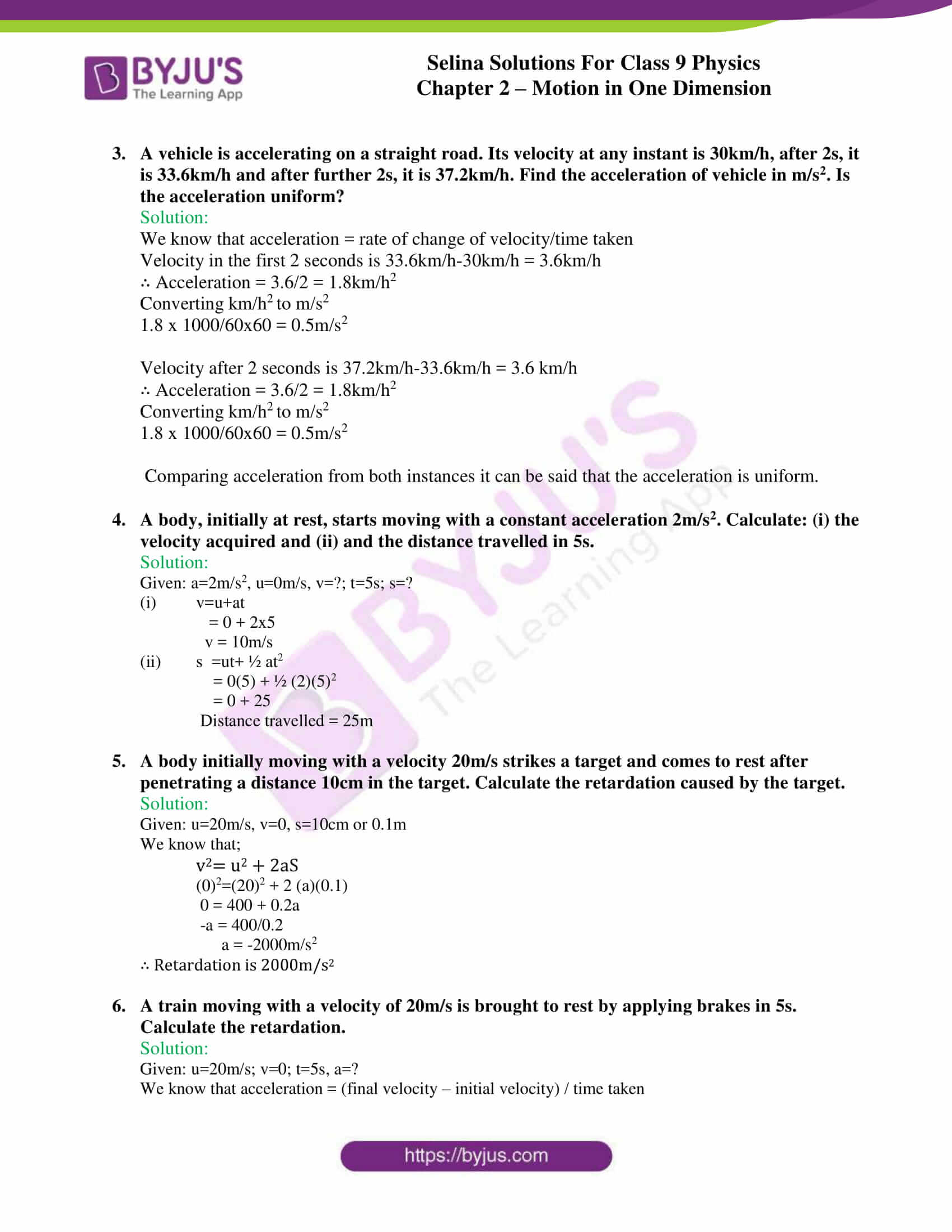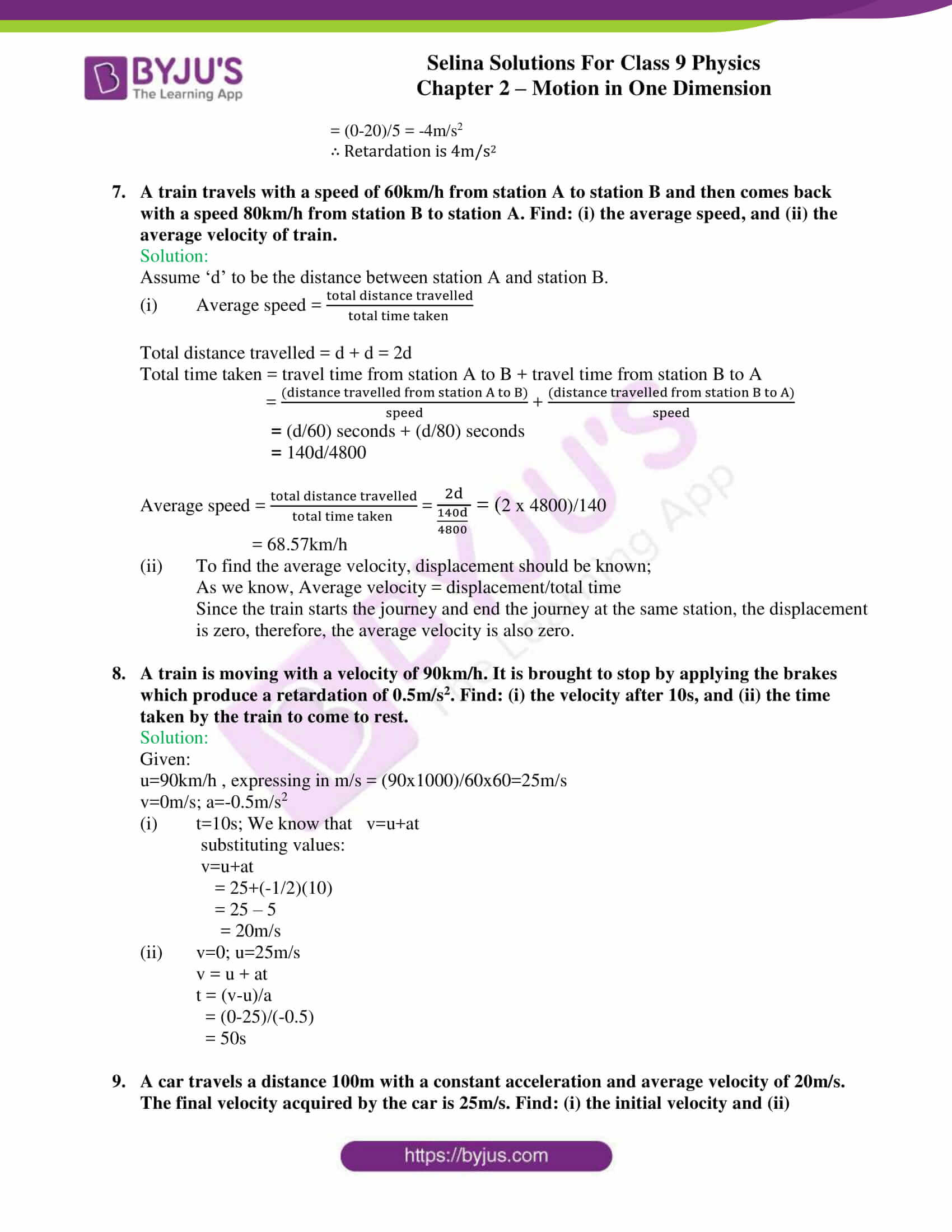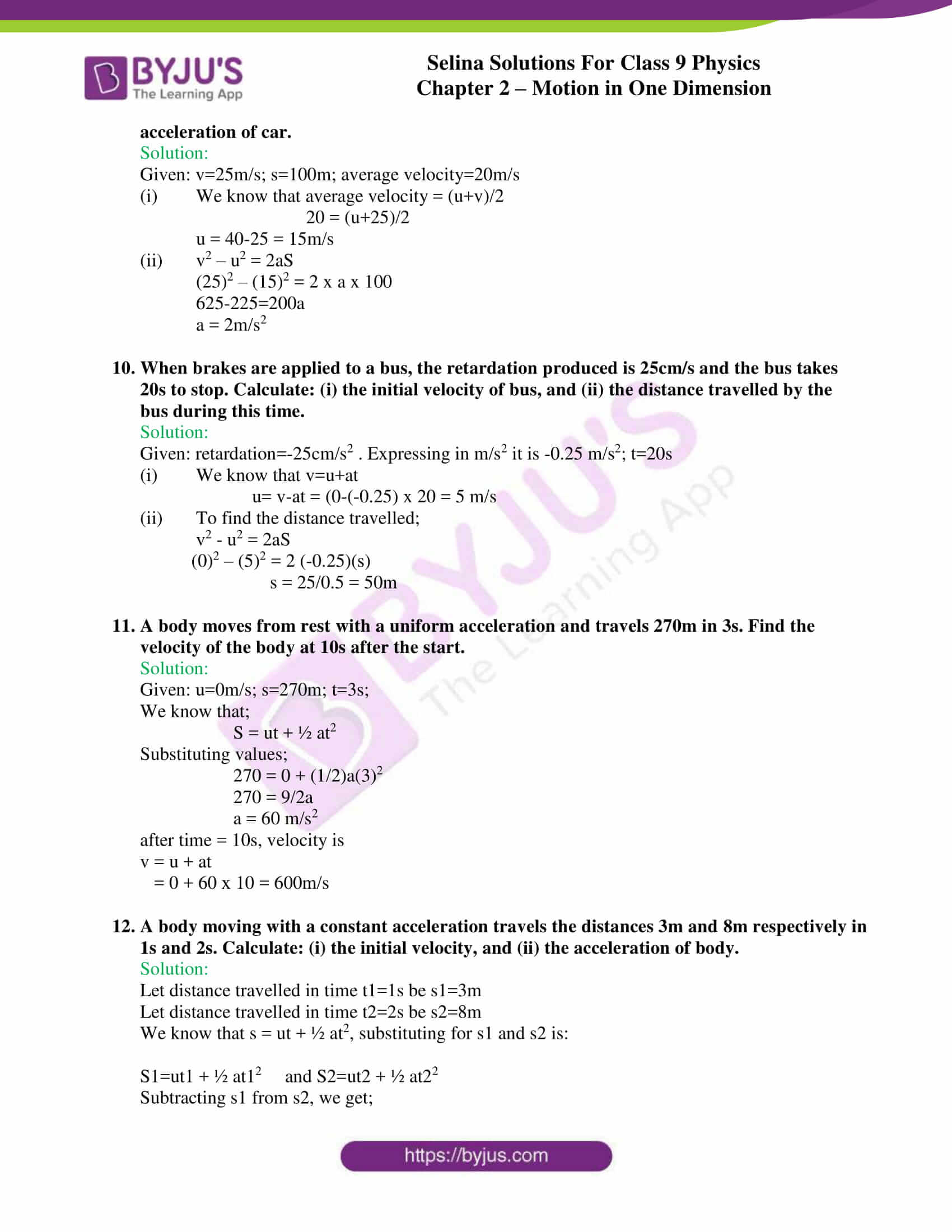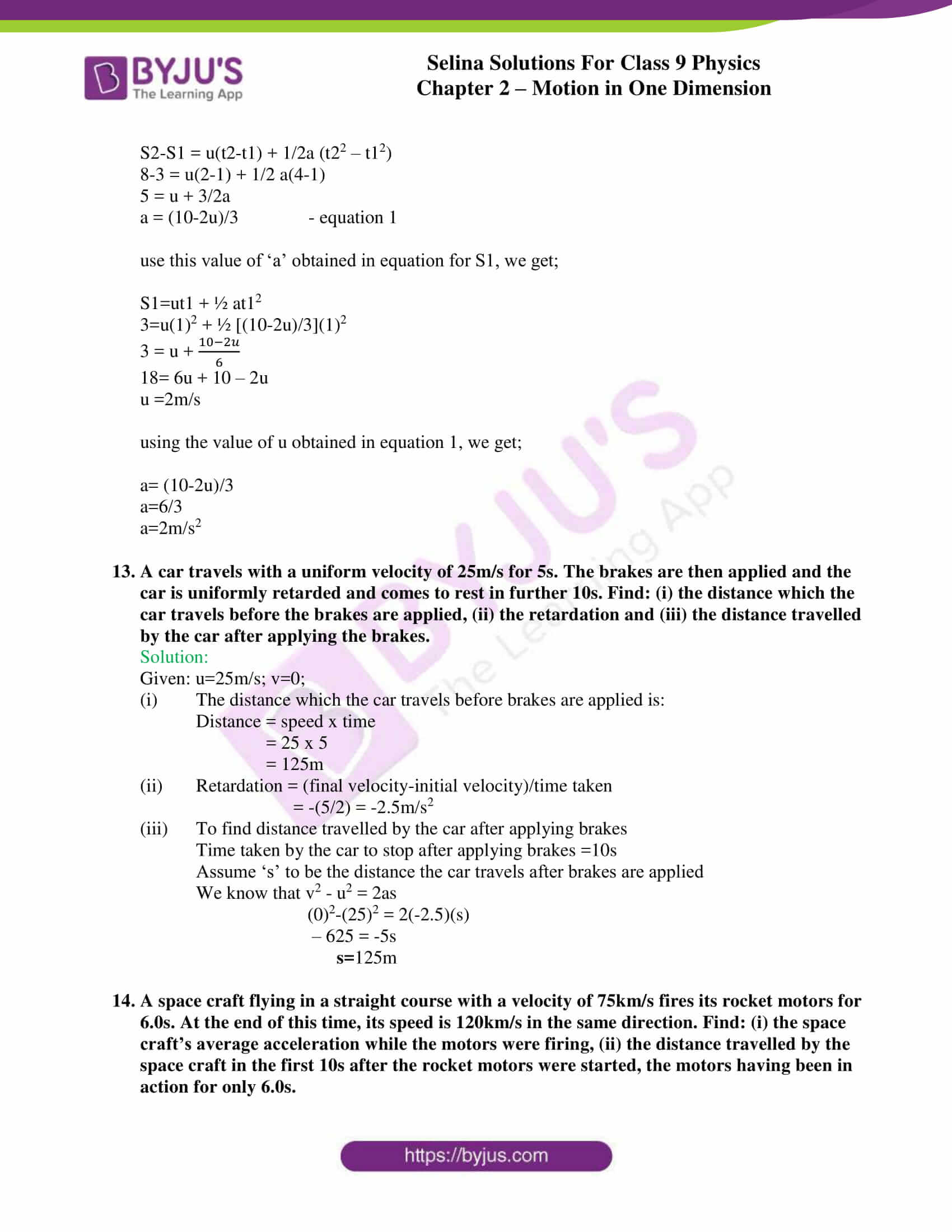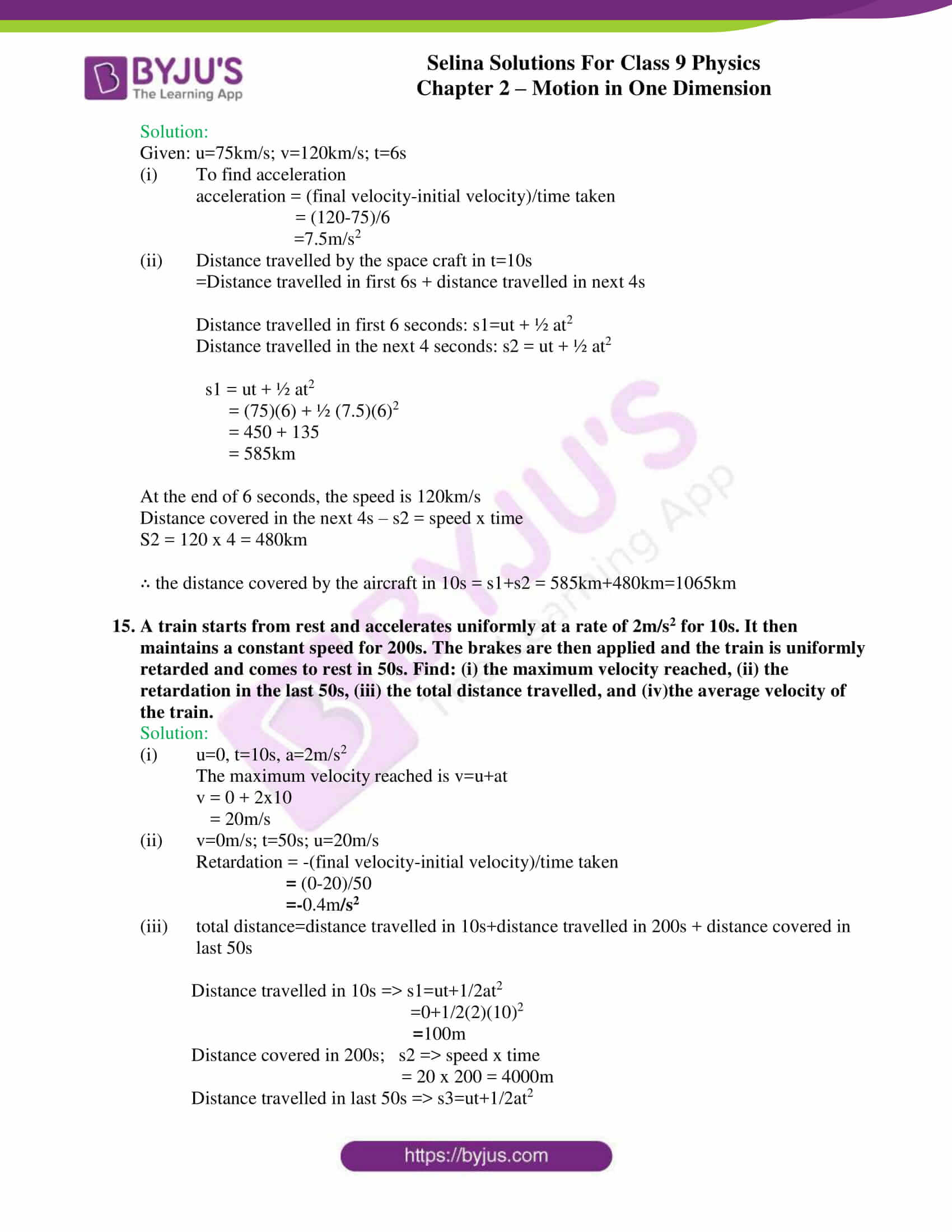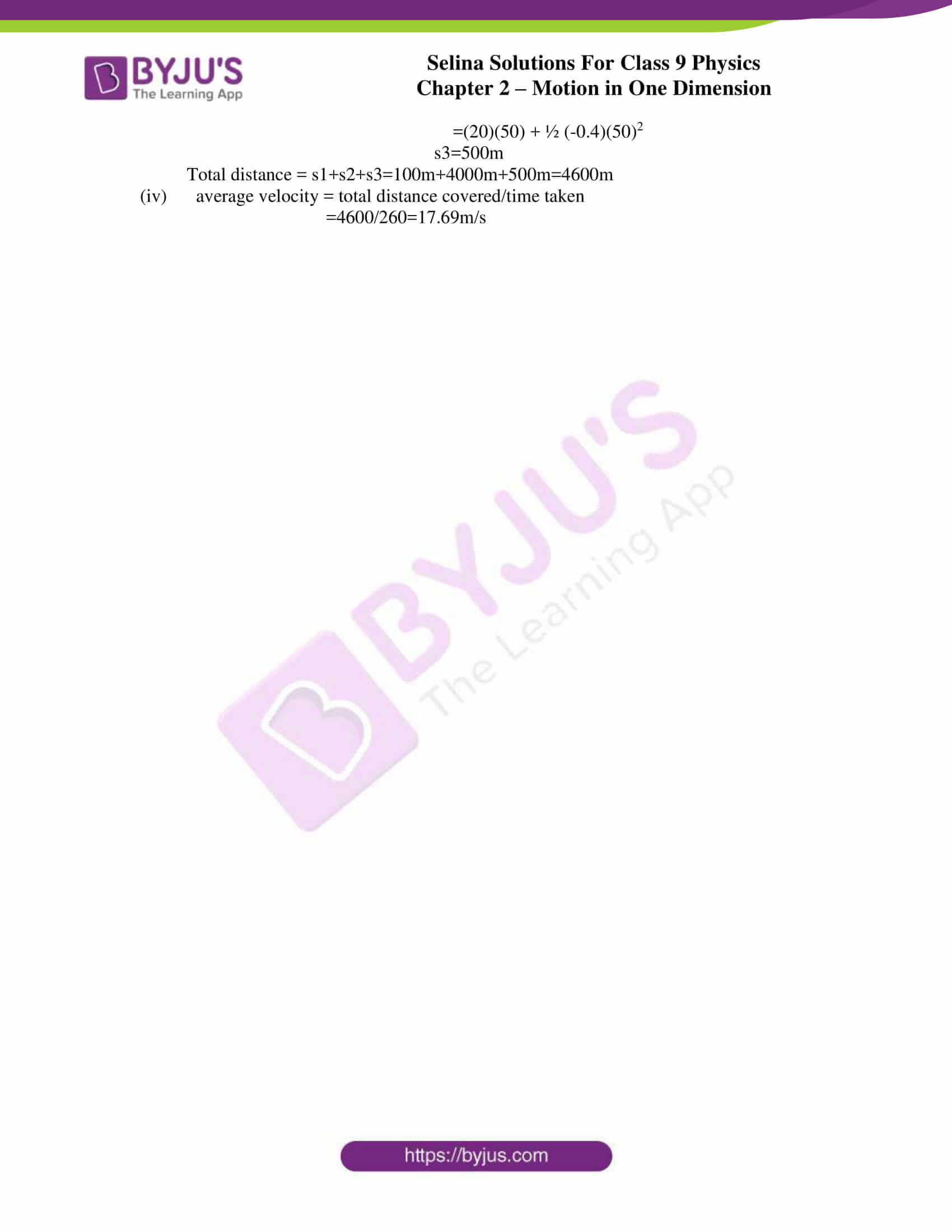## Selina Solutions for Class 9 Physics Chapter 2 – Motion in One Dimension

ICSE Class 9 Physics 2 – Motion in One Dimension provides a clear understanding to students about the scalar and vector quantities, the different aspects involved in motion regarding one dimension.

After having studied about the various aspects of measurements and experimentation in the previous chapter which sheds light on physical quantities, their corresponding units, symbols, instruments used to measure various dimensions, this chapter discusses the different examples of scalar and vector quantities, distance, displacement and velocity, acceleration, retardation, different graphs, etc.

The chapter also enlightens students about the various equations, its derivations and simple numerical problems towards the end, thereby covering practical aspects of the topics.

List of subtopics covered in Chapter 2 – Motion in One Dimension:

 Number Subtopic 2.1 Scalar and vector quantities 2.2 Rest and motion 2.3 Distance and displacement 2.4 Speed and velocity 2.5 Acceleration and retardation 2.6 Displacement- time graph 2.7 Velocity-time graph 2.8 Acceleration-time graph 2.9 Motion under gravity

List of Exercise

 Name of the exercise Number of questions Page number Exercise-2A 49 35 Exercise-2B 33 47 Exercise-2C 20 55

## Selina Solutions for Class 9 Physics Chapter 2 – Motion in One Dimension

The chapter provides details to some terms related to motion such as different types of quantities:

• Scalar
• Vector

The various aspects of rest and motion, distance and displacement- the difference between the two quantities, their representation etc. Speed and velocity are concepts that are often correlated, through this chapter we understand their difference and solve numerical problems on it. Graphical representation of linear motion through – velocity-time graph, displacement-time graph, acceleration-time graph are also depicted along with examples.

Towards the end, the chapter also discusses the equations of uniformly accelerated motion, their derivations through different methods, etc.

### Key Features of Selina Solutions for Class 9 Physics 2 – Motion in One Dimension

• Selina solutions is a student-friendly study tool
• It is easily accessible and framed with the use of simple terms
• Solutions to numerical problems are provided with complete explanation
• Use of graphs, wherever necessary to aid explanation
• Use of tables, wherever necessary.
• Solutions are developed according to the latest syllabus prescribed by the C.I.S.C.E.

## Frequently Asked Questions on Motion in one dimension

### When is a body said to be at rest?

A body is referred to be in a state of rest or stationary when there is no change in the position of the body with regards to its immediate surroundings.

### When is a body said to be in motion?

A body is referred to be in a state of motion when there are changes in the position of the body with regards to its immediate surroundings.

### What do you mean by motion in one direction?

Motion in one direction with reference to a body is when the body moves in a straight line path only.

### Define displacement.

Displacement is the shortest distance from the initial to the final position of the body – magnitude wise. The direction of displacement is considered from the initial position to the final position. The S.I unit of displacement is the metre (m).

### Define velocity.

Velocity can be defined as the distance covered per second by a body in a particular direction. The S.I. unit of velocity is metre/second (m/s).

### Define speed?

Speed can be defined as the rate of change of distance with regards to time. The S.I. unit of time is metre/second (m/s).

### When is the instantaneous speed the same as the average speed?

The instantaneous speed is the same as the average speed when the acceleration of the body is zero. In order for the acceleration to be zero, neither the speed nor the direction changes.

### Define acceleration.

Acceleration can be defined as the rate of change of velocity with time. It can also be defined as the increase in velocity per second. The S.I unit of acceleration is meter per second square or ms-2.

### What is meant by the term retardation?

Retardation can be defined as the decrease in the velocity per second. Since negative acceleration is retardation, its S.I. unit is the same as acceleration.